CGABC Chenguang Career from Zero [A] to Hero [Z] 2022-12-24T07:16:06.044Z https://cgabc.xyz/ Gavin Gao Hexo When SLAM meets XR and Robotics https://cgabc.xyz/posts/eceda3cc/ 2022-12-23T16:00:00.000Z 2022-12-24T07:16:06.044Z When SLAM meets XR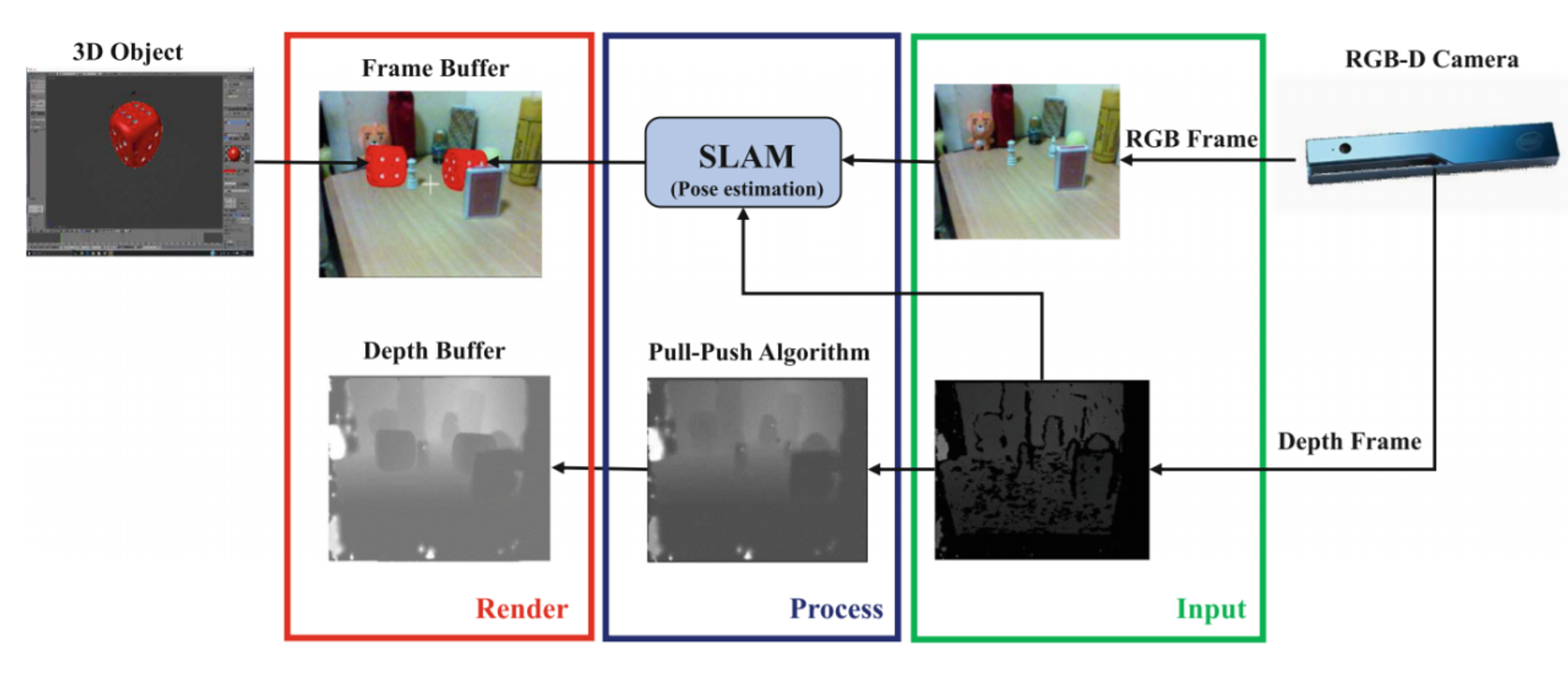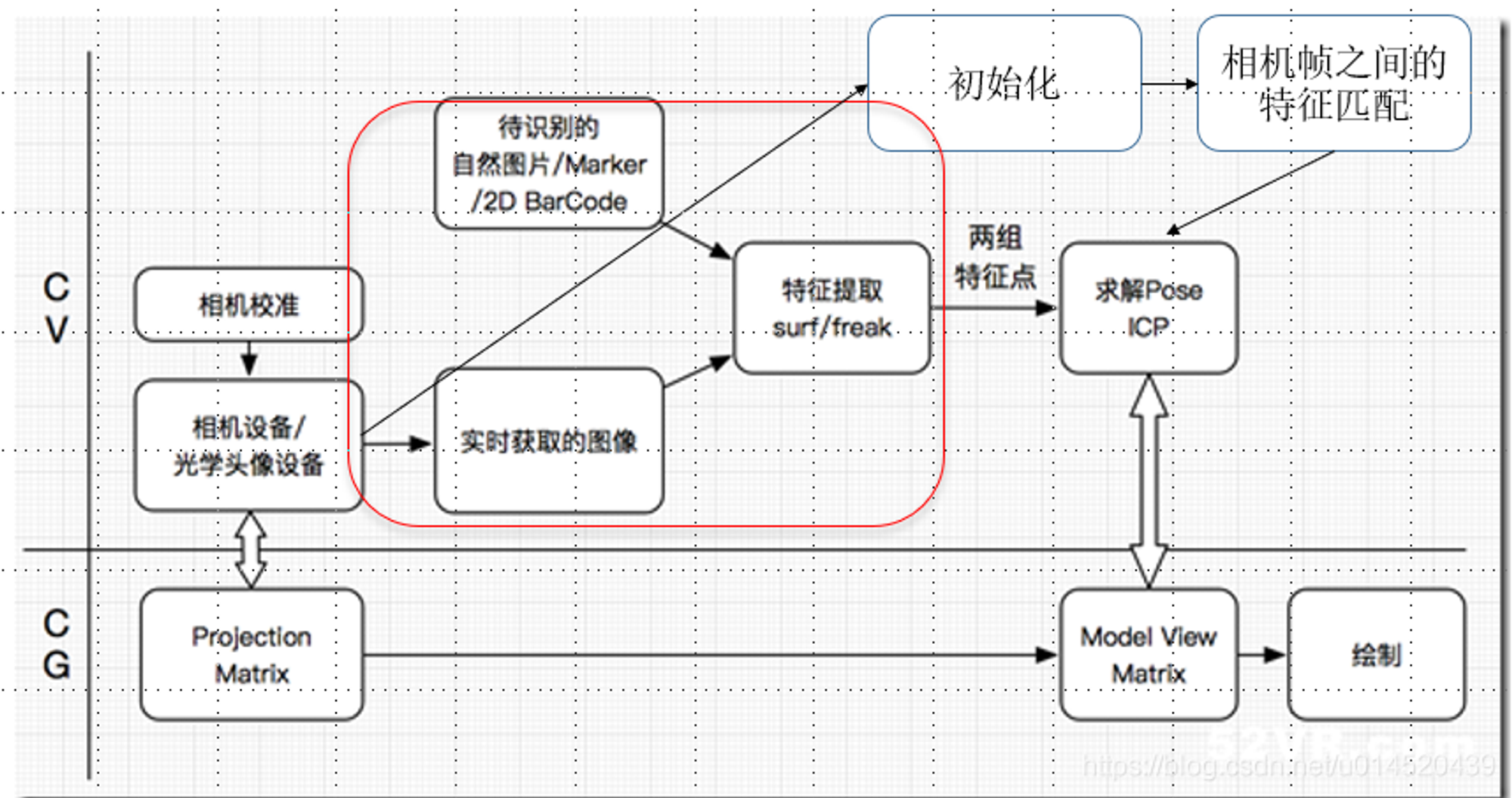# When SLAM meets Robotics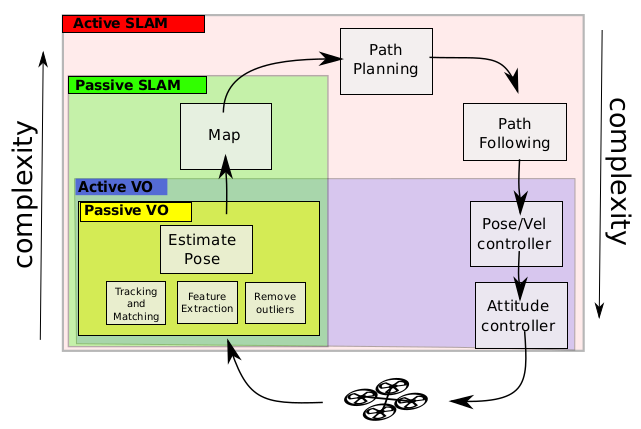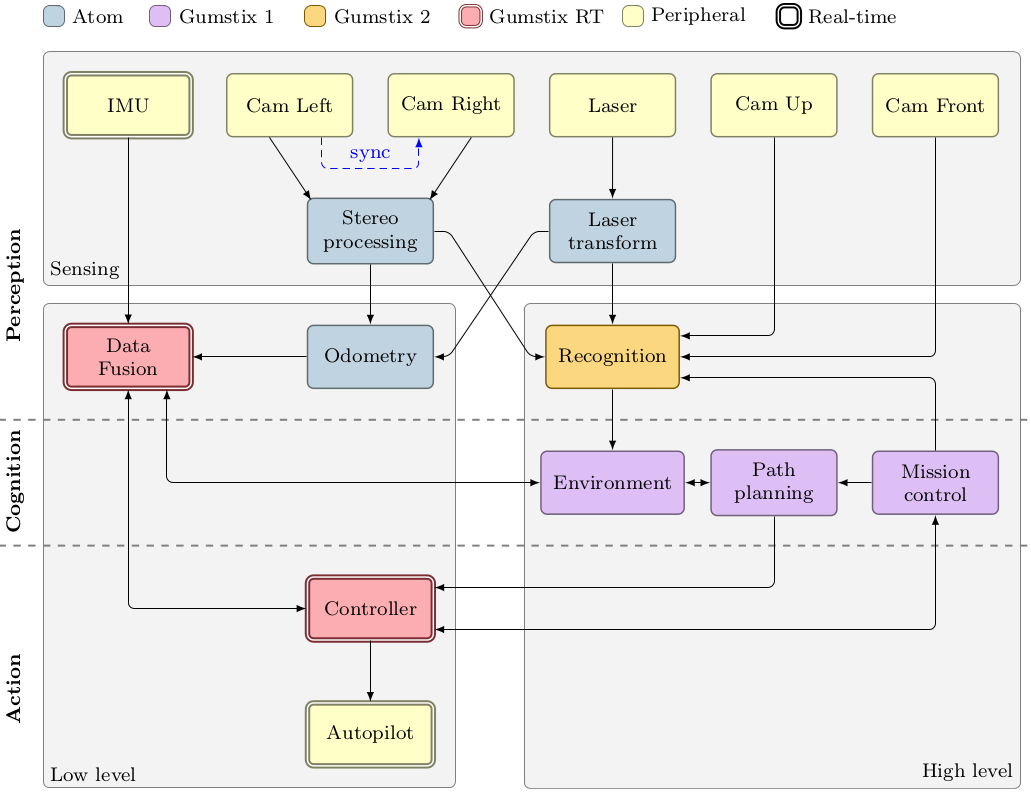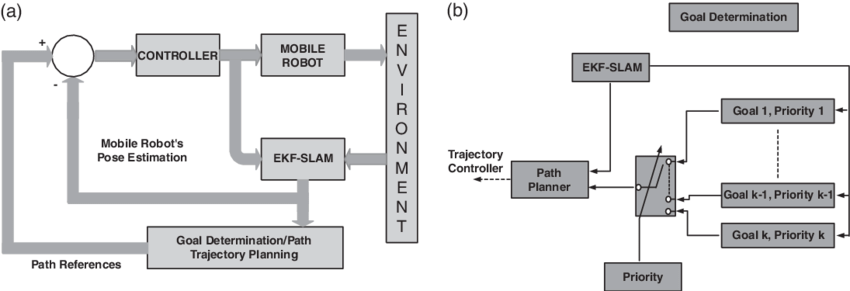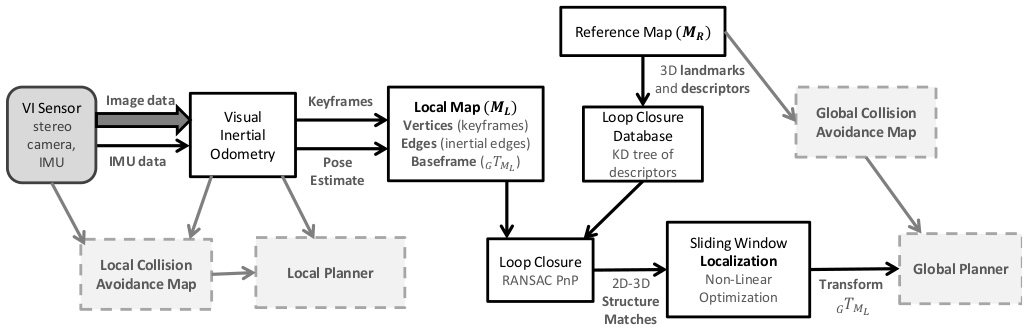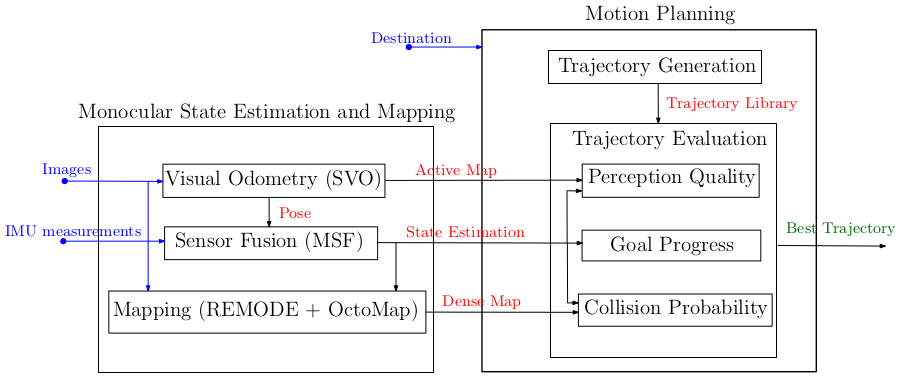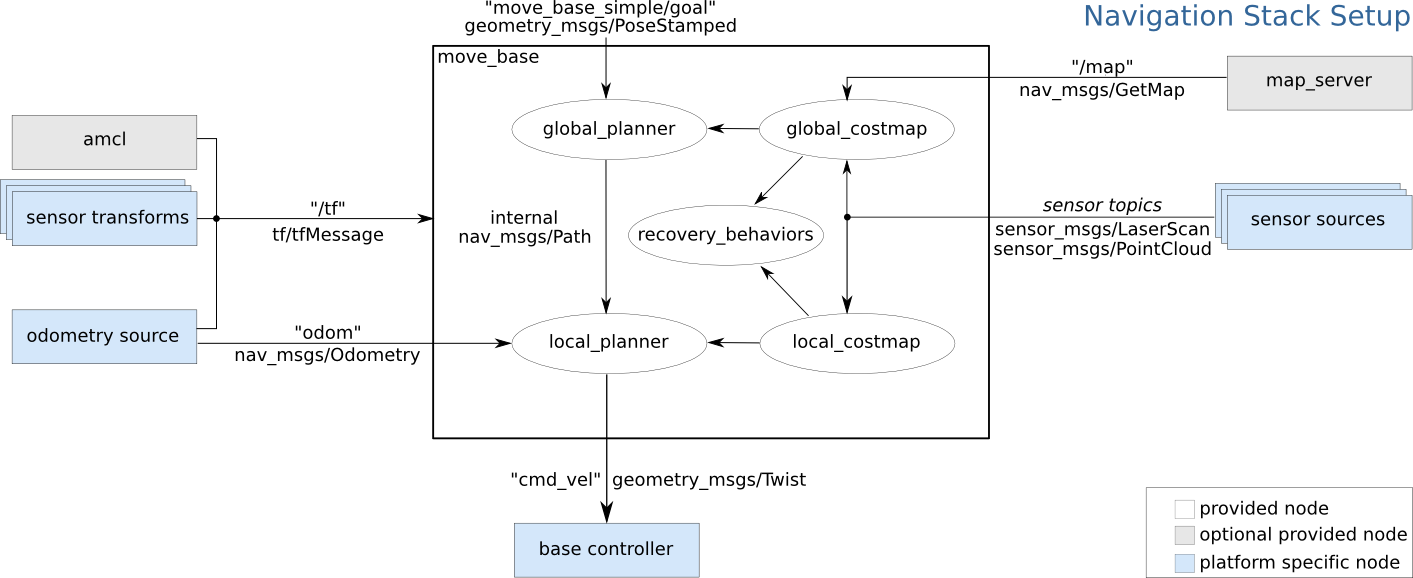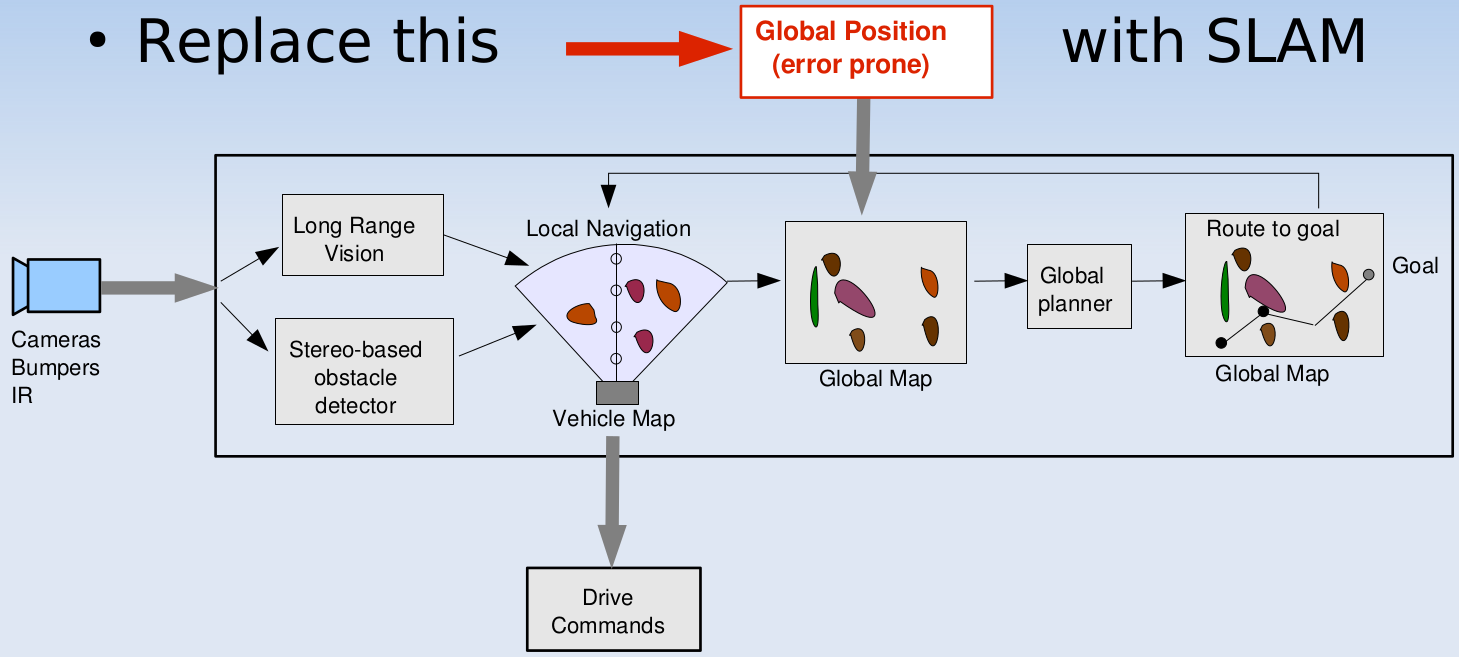]]>
<h1 id="when-slam-meets-xr">When SLAM meets XR</h1> <p align="center"> <img src="/img/post/slam_app/slam_xr_00.png" style="width:100%;"> <im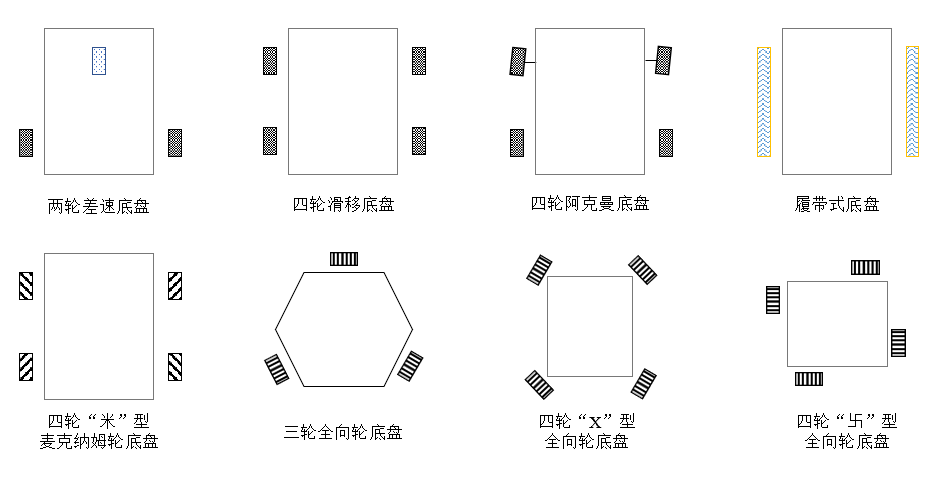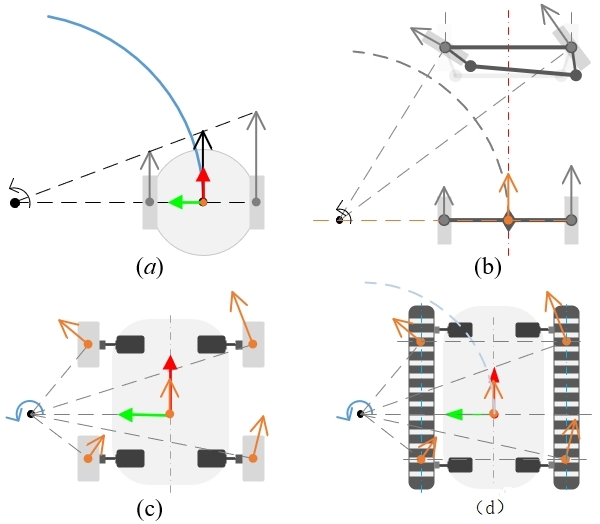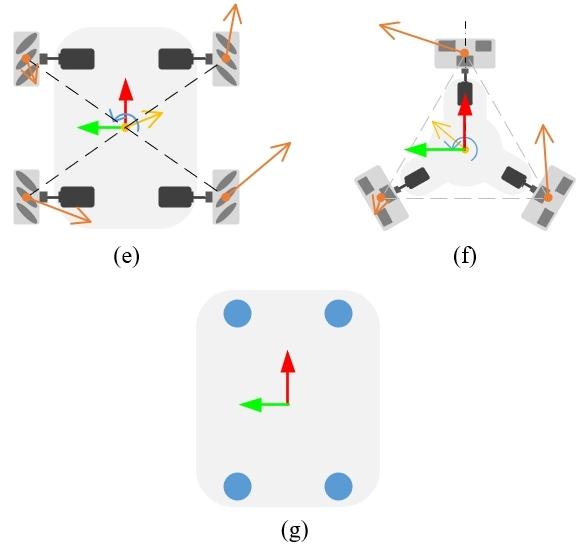ROS 中运动学分析为正解（Forward kinematics）和逆解（InverseKinematics）两种。

• 正解 或 正运动学模型（forward kinematicmodel）：是将获得的机器人底盘速度指令 /cmd_vel转化为每个车轮的实际速度。
• 逆解 或 逆运动学模型（inverse kinematicmodel）：是根据电机编码器获得的每个车轮速度计算出机器人底盘速度，从而实现航迹推算。

# 两轮差速运动模型(Differential Drive robot)

ROS自带的DWA路径规划算法比较适合，why？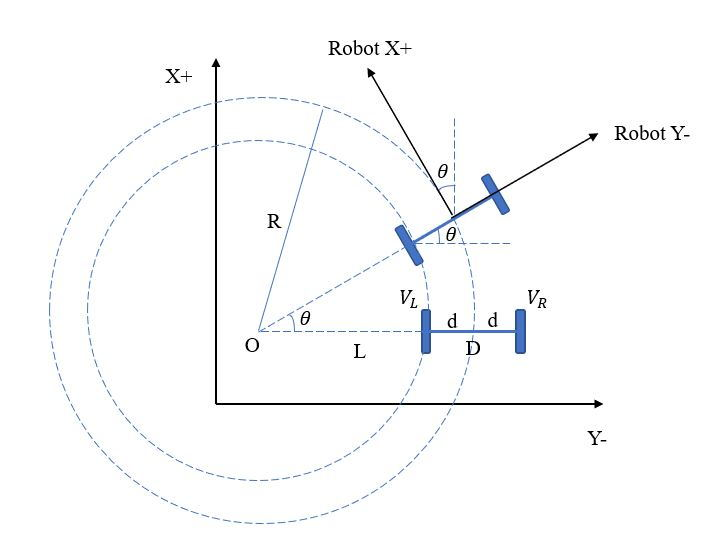## Forward Kinematic Model

$v = \frac{v_l + v_r}{2}$

$\dot P =\begin{bmatrix} c & -s & 0 \\ s & c & 0 \\ 0 & 0& 1 \end{bmatrix}\begin{bmatrix} v_x \\ v_y \\ v_\theta \end{bmatrix}$

$P_1 = P_0 + \dot P \cdot \Delta t$

# 全向移动底盘（omnidirectionalwheel robot）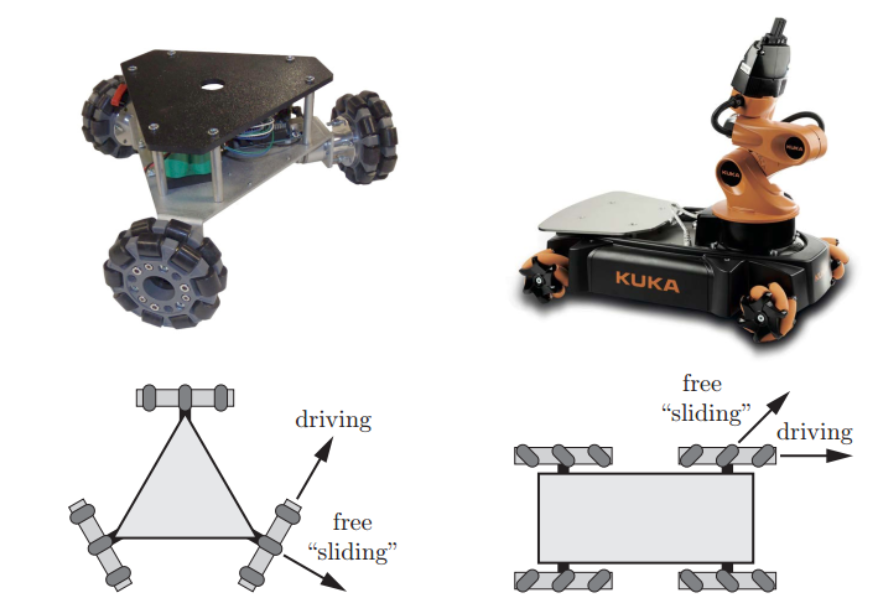# Reference

]]>
<h1 id="overview-1">Overview <sup id="fnref:1" class="footnote-ref"><a href="#fn:1" rel="footnote"><span class="hint--top hint--rounded" ari
On-Manifold Optimization: Local Parameterization https://cgabc.xyz/posts/cfb7b6d6/ 2022-09-04T11:30:00.000Z 2022-09-04T11:36:15.954Z Overview

### Manifold Space vs TangentSpace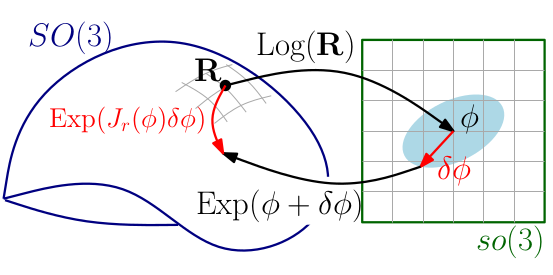## Jacobian w.r.t Error State

### Jacobian w.r.t ErrorState vs True State

According2.4,

The idea is that for a $$x \in N$$the function $$g(\delta) := f (x \boxplus\delta)$$ behaves locally in $$0$$ like $$f$$ does in $$x$$. In particular $$\|f(x)\|^2$$ has a minimum in $$x$$ if and only if $$\|g(\delta)\|^2$$ has a minimum in $$0$$. Therefore finding a local optimum of$$g$$, $$\delta = \arg \min_{\delta}\|g(\delta)\|^2$$ implies $$x \boxplus\delta = \arg \min_{\xi} \|f(\xi)\|^2$$.

$f(x \boxplus \delta)=f(x)+J_x\delta+\mathcal{O}\left(\|\delta\|^2\right)$

where

$J = \left. \frac{\partial f(x \boxplus \delta)}{\partial \delta}\right|_{\delta=0}\quad \longleftrightarrow \quadJ = \left. \frac{\partial f(x)}{\partial x} \right|_{x}$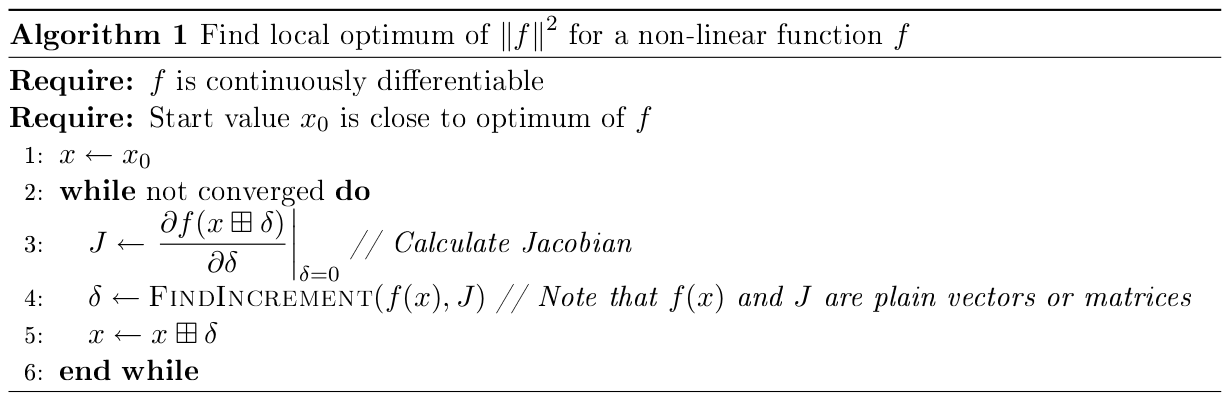### ESKF6.1.1: Jacobian computation

$\left.\mathbf{H} \triangleq \frac{\partial h}{\partial \delta\mathbf{x}}\right|_{\mathbf{x}}=\left.\left.\frac{\partial h}{\partial\mathbf{x}_t}\right|_{\mathbf{x}} \frac{\partial \mathbf{x}_t}{\partial\delta \mathbf{x}}\right|_{\mathbf{x}}=\mathbf{H}_{\mathbf{x}}\mathbf{X}_{\delta \mathbf{x}}$

• $$x_t$$: true state
• $$x$$: normal state
• $$\delta x$$: error state

lifting and retraction: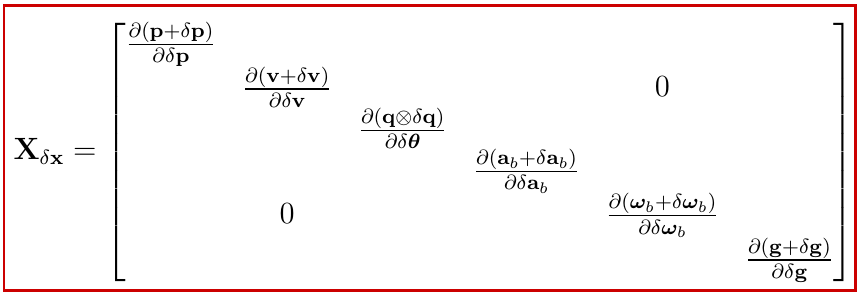$\left.\mathbf{X}_{\delta \mathbf{x}} \triangleq \frac{\partial\mathbf{x}_t}{\partial \delta\mathbf{x}}\right|_{\mathbf{x}}=\left[\begin{array}{ccc}\mathbf{I}_6 & 0 & 0 \\0 & \mathbf{Q}_{\delta \boldsymbol{\theta}} & 0 \\0 & 0 & \mathbf{I}_9\end{array}\right]$

the quaternion term

\begin{aligned}\left.\mathbf{Q}_{\delta \boldsymbol{\theta}} \triangleq\frac{\partial(\mathbf{q} \otimes \delta \mathbf{q})}{\partial \delta\boldsymbol{\theta}}\right|_{\mathbf{q}}&=\left.\left.\frac{\partial(\mathbf{q} \otimes \delta\mathbf{q})}{\partial \delta \mathbf{q}}\right|_{\mathbf{q}}\frac{\partial \delta \mathbf{q}}{\partial \delta\boldsymbol{\theta}}\right|_{\delta \hat{\boldsymbol{\theta}}=0} \\&=\left.\left.\frac{\partial\left([\mathbf{q}]_L \delta\mathbf{q}\right)}{\partial \delta \mathbf{q}}\right|_{\mathbf{q}}\frac{\partial\left[\begin{array}{c}1 \\\frac{1}{2} \delta \boldsymbol{\theta}\end{array}\right]}{\partial \delta\boldsymbol{\theta}}\right|_{\hat{\delta}=0} \\&=[\mathbf{q}]_L \frac{1}{2}\left[\begin{array}{lll}0 & 0 & 0 \\1 & 0 & 0 \\0 & 1 & 0 \\0 & 0 & 1\end{array}\right]\end{aligned}

### Least Squares on a Manifold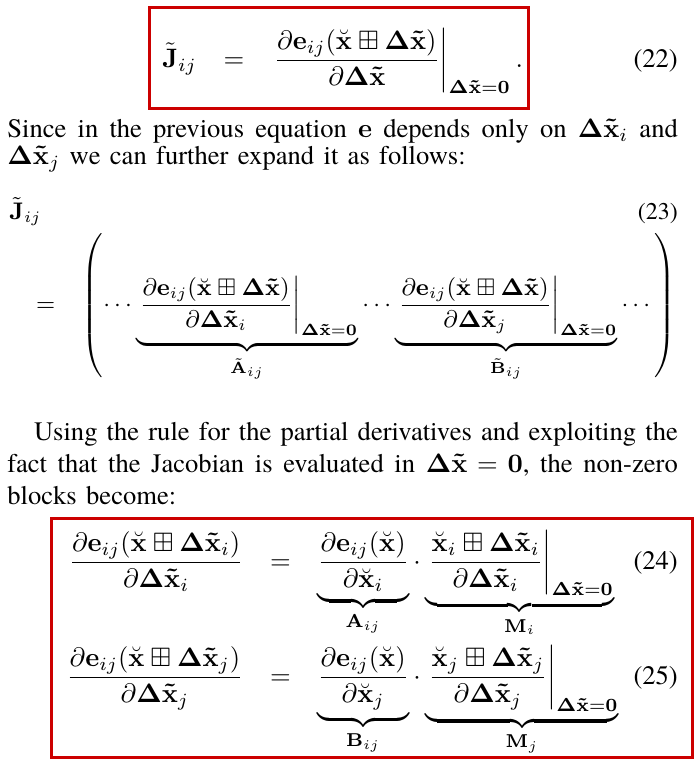## LocalParameterization in Ceres Solver

### Plus

Retraction

$\boxplus(x, \Delta)=x \operatorname{Exp}(\Delta)$

### ComputeJacobian

global w.r.t local

$J_{GL}= \frac{\partial x_G}{\partial x_L}= D_2 \boxplus(x, 0)= \left. \frac{\partial \boxplus(x, \Delta)}{\partial \Delta}\right|_{\Delta = 0}$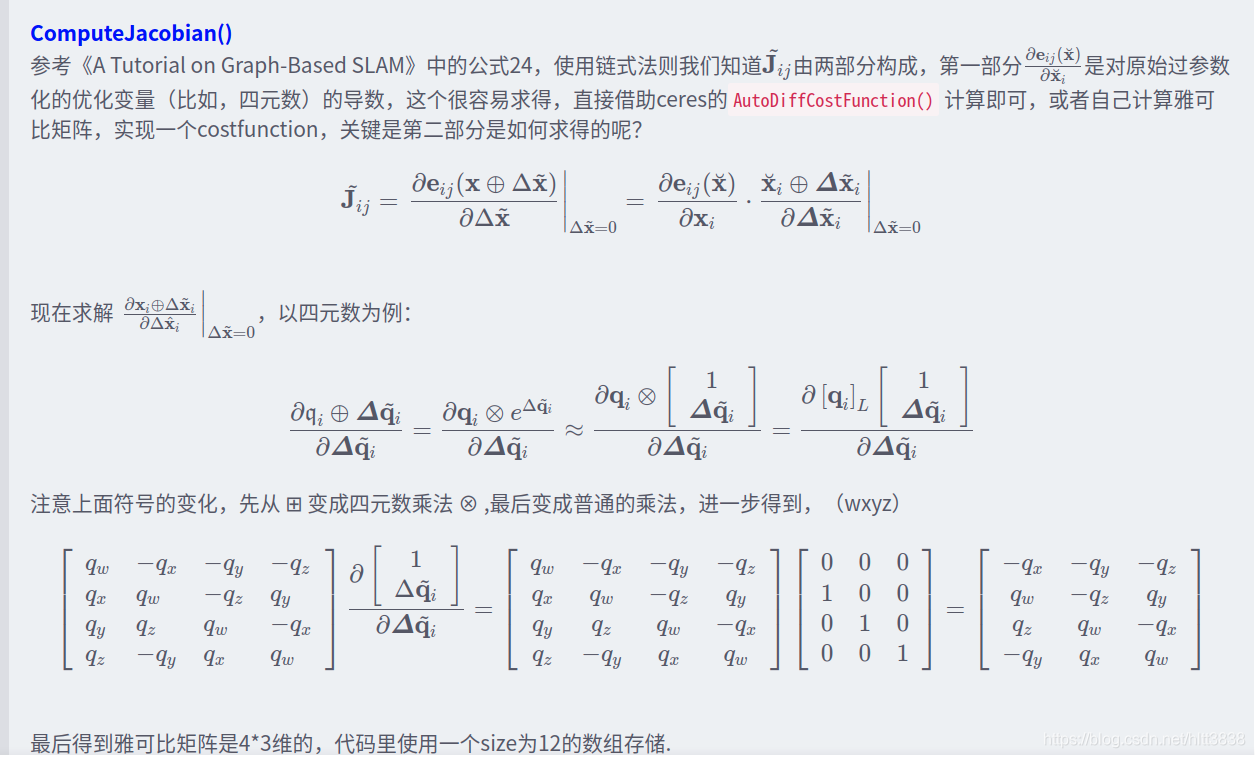#### $$r$$ w.r.t $$x_{L}$$

ceres::CostFunction 处提供 residuals 对 Manifold上变量的导数

$J_{rG} = \frac{\partial r}{\partial x_G}$

$J_{rL}= \frac{\partial r}{\partial x_L}= \frac{\partial r}{\partial x_G} \cdot J_{GL}$

### Sub Class

• QuaternionParameterization
• EigenQuaternionParameterization

### 自定义QuaternionParameterization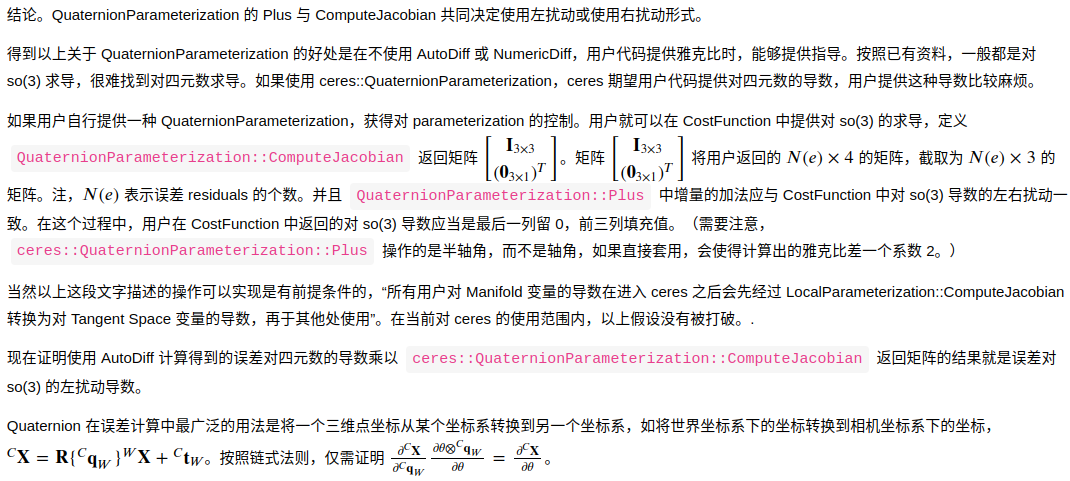### Summary

• QuaternionParameterization 的 Plus 与 ComputeJacobian共同决定使用左扰动或使用右扰动形式

Quaternion in Eigen

Quaternion in Ceres Solver

• order: wxyz
• Ceres Solver 中 Quaternion 是 Hamilton Quaternion，遵循 Hamilton乘法法则
• 矩阵 raw memory 存储方式是 Row Major

# Reference

1. A Framework for Sparse,Non-Linear Least Squares Problems on Manifolds↩︎
2. Quaternion kinematics forthe error-state Kalman filter, Joan Solà↩︎
3. A Tutorial on Graph-BasedSLAM ↩︎
4. http://ceres-solver.org/nnls_modeling.html#localparameterization↩︎
5. [ceres-solver]From QuaternionParameterization to LocalParameterization 😄↩︎
]]>
<h2 id="overview">Overview</h2> <h3 id="manifold-space-vs-tangent-space">Manifold Space vs Tangent Space</h3> <p align="center"> <img src="/
Mapillary API at a Glance https://cgabc.xyz/posts/ec7b8646/ 2022-07-23T16:00:00.000Z 2022-08-26T12:03:32.800Z Embed images

The iframe tag below would show a 640x480px image with ID550092599700936.

# Reference

]]>
<h1 id="embed-images-1">Embed images <sup id="fnref:1" class="footnote-ref"><a href="#fn:1" rel="footnote"><span class="hint--top hint--roun
Observability and Inconsistency in a Nutshell https://cgabc.xyz/posts/881febbe/ 2022-07-15T16:00:00.000Z 2022-08-26T12:03:32.808Z [TOC]

# Overview

What is observability ?

In control theory, observability is a measure for how well internalstates of a system can be inferred by knowledge of its externaloutputs.

What is consistency ?

A recursive estimator is consistent when the estimation errors arezero-mean and have covariance matrix equal to that reported by theestimator.

Observability $$\longrightarrow$$Consistency

Mismatch (actual vs true) in observability $$\longrightarrow$$ Inconsistency

VINS observability properties $$\longrightarrow$$ estimator inconsistency

# Basics

## Nullspace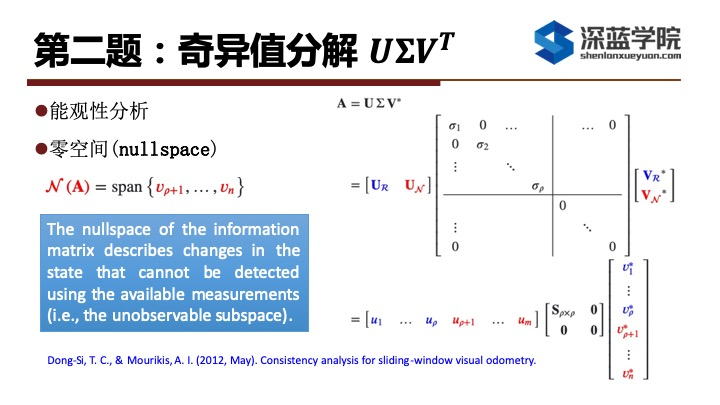## Lie Derivative

\begin{aligned}h(x):& \; R^n \rightarrow R \\f(x):& \; R^n \rightarrow R^n \\g(x):& \; R^n \rightarrow R^n\end{aligned}

$L_f h = \nabla h \cdot f = \left( \frac{\partial h}{\partial x}\right)^T f$

$$L_g L_f h$$ 结果依然是个标量

$L_g L_f h = L_g (L_f h)= \nabla(L_f h) g= \left( \frac{\partial (L_f h)}{\partial x} \right)^T g= \left( \frac{\partial \left( (\frac{\partial h}{\partial x})^T f\right)}{\partial x} \right)^T g$

# Observability Analysis

• what: 控制理论中的可观察性（observability）是指系统可以由其外部输出推断其内部状态的程度。

• why:为了能让系统不可观的维度与真实系统一致，从而提高系统精度

• how:通过计算可观性矩阵，分析其零空间的秩，来分析系统哪些状态维度可观/不可观；可观性矩阵对应系统可观测的维度，零空间对应系统不可观的维度

## Unobservable DoF (GaugeFreedom) in SLAM

• Mono vSLAM: 7
• 6 DoF 绝对位姿 + 尺度
• Stereo vSLAM: 6
• 6 DoF 绝对位姿
• Mono + IMU SLAM: 4
• 3 DoF 绝对位置 + 绝对yaw角
• roll 和 pitch由于重力的存在而可观，尺度因子由于加速度计的存在而可观

## Observability Matrix

Discrete state space equations of nonlinear systems (linearizedwithout considering noise) is

$\begin{cases} x_{k+1} = \Phi_k x_k \\ y_k = H_k x_k \end{cases}$

according to the Lie derivative, theobservability matrix is

$\mathbf{\mathcal{O}} \left(\mathbf{x}^{\star}\right) =\left[ \begin{array}{c} \mathbf{H}_{1} \\ \mathbf{H}_{2} \boldsymbol{\Phi}_{2,1} \\ \vdots \\ \mathbf{H}_{k} \boldsymbol{\Phi}_{k, 1} \end{array}\right]$

then, the unobservable dimensions of the system are

$\text{rank}(N(\mathcal{O}))$

### ObservabilityMatrix vs Hessian(Information) Matrix

for the monocular VO based on optimization methods, the dimension ofnull space of the Hessian (Information) matrix $$H$$ is 7, that is, the unobservabledimensions are

$\text{rank}(N(H)) = 7$

$J^T J \Delta x = - J^T r \quad \longrightarrow \quad H \Delta x = b$

What is the relationship between the Hessian matrix $$H$$ and the observability matrix $$\mathcal{O}$$ in the optimization basedVO/VIO ?

• paper: Observability-Based Guidance and SensorPlacement (Chapter 2 - OBSERVABILITY MEASURES) > As anote, the measurement Jacobian, $$dY$$,is equivalent to the observability matrix, $$d\mathcal{O}$$, evaluated at a nominalstate, $$x_0$$.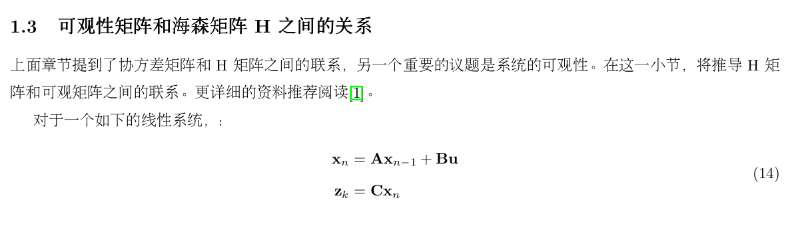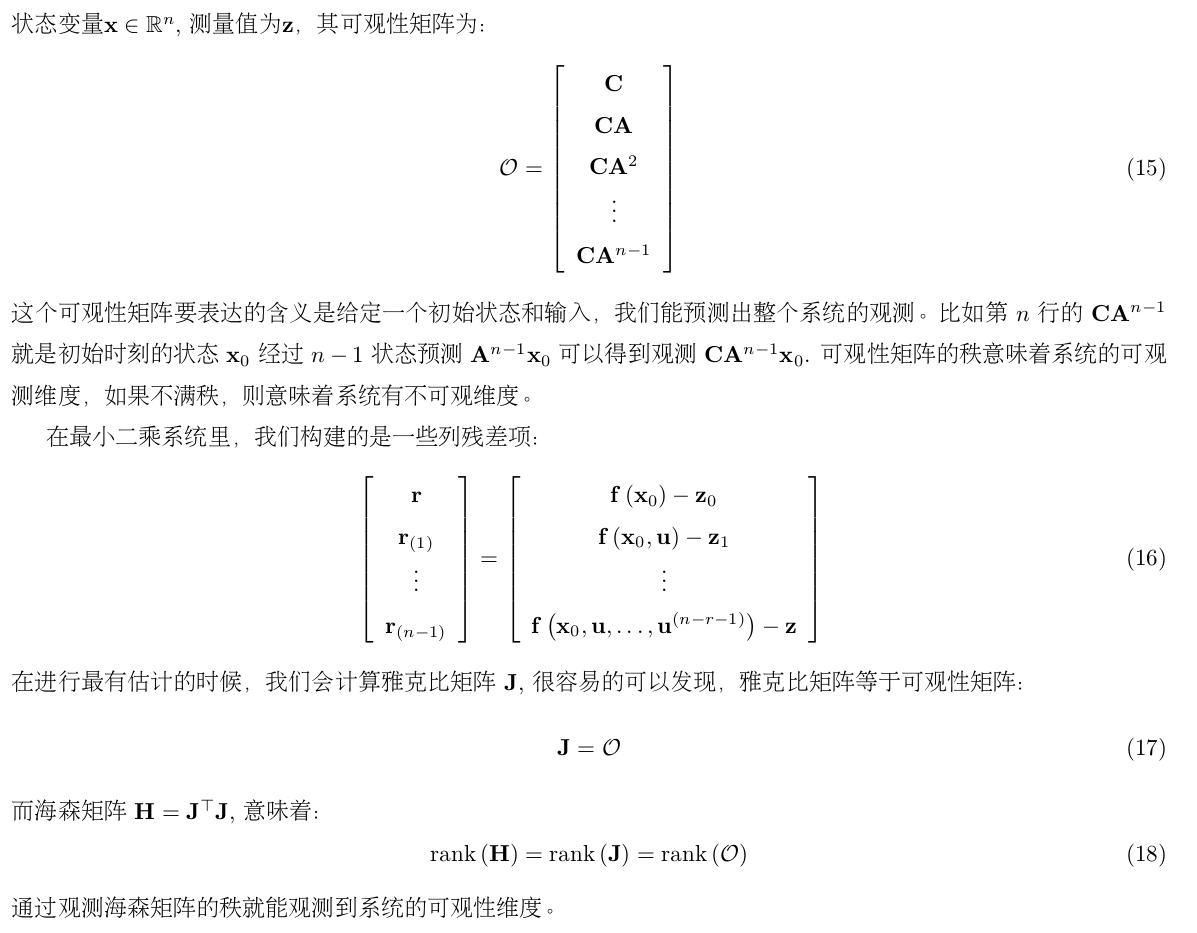## NEES (normalizedestimation error squared)

• NEES closer to 6 for VIO

# Inconsistency of Estimator

a state estimator is consistent if the estimation errors (i) arezero-mean, and (ii) have covariance matrix smaller or equal to the onecalculated by the filter.

## Degeneracy(Insufficient Restraint) / Inconsistency in SLAM

### Motion

• constant acceleration
• pure translation

## Maintain(Solve)Consistency(Inconsistency)

paper:

• VINS on Wheels
• Odometry measurements
• Planar-motion constraints

### FEJ (First-EstimateJacobians)

• paper: A First-Estimates Jacobian EKF for ImprovingSLAM Consistency

• estimation from the first time

to ensure that the state transition and Jacobian matrices areevaluated at correct linearization points such that the aboveobservability analysis will hold true

FEJ算法：不同残差对同一个状态求雅克比时，线性化点必须一致，这样就能避免零空间退化而使得不可观变量变得可观。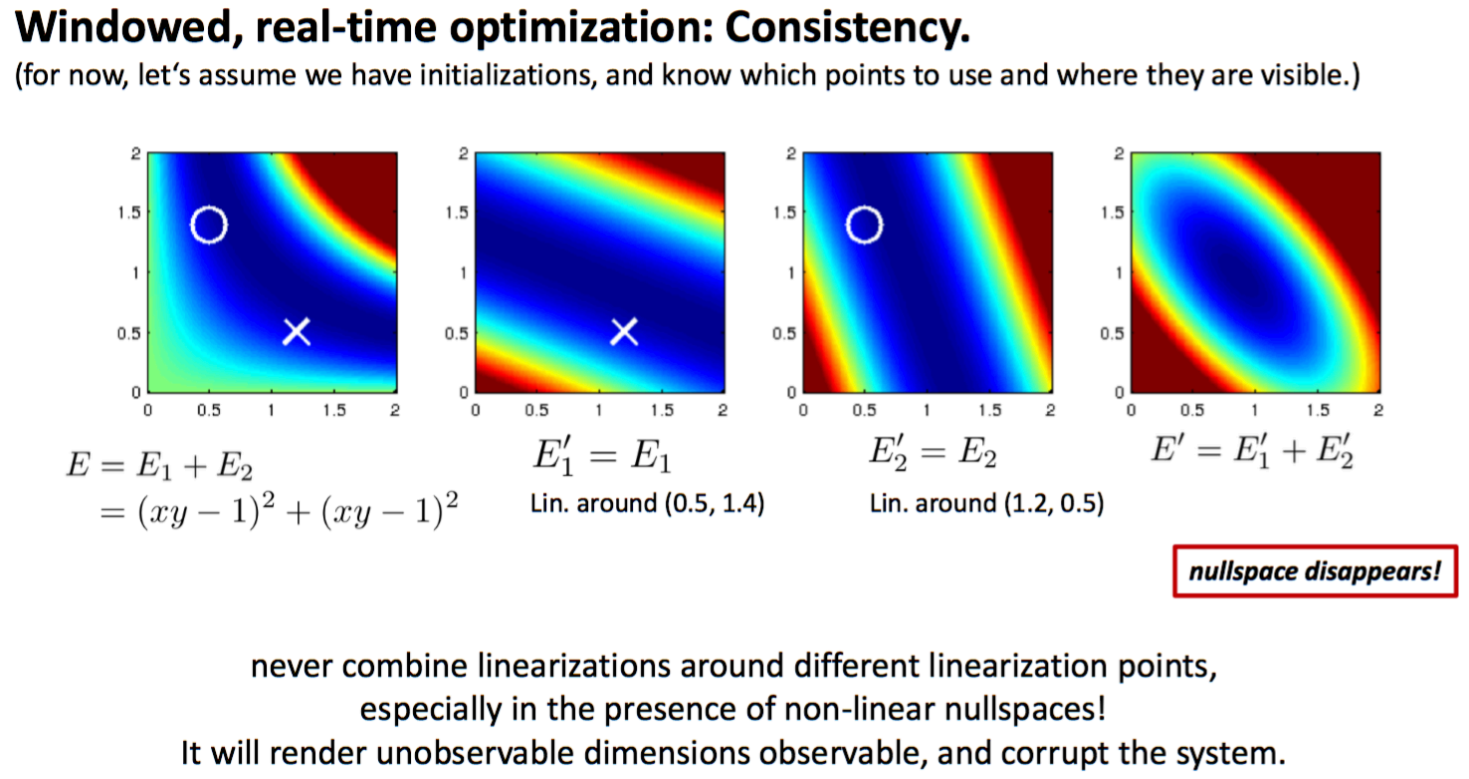app:

ref:

TODO

### Observability Constraint(OC)-VINS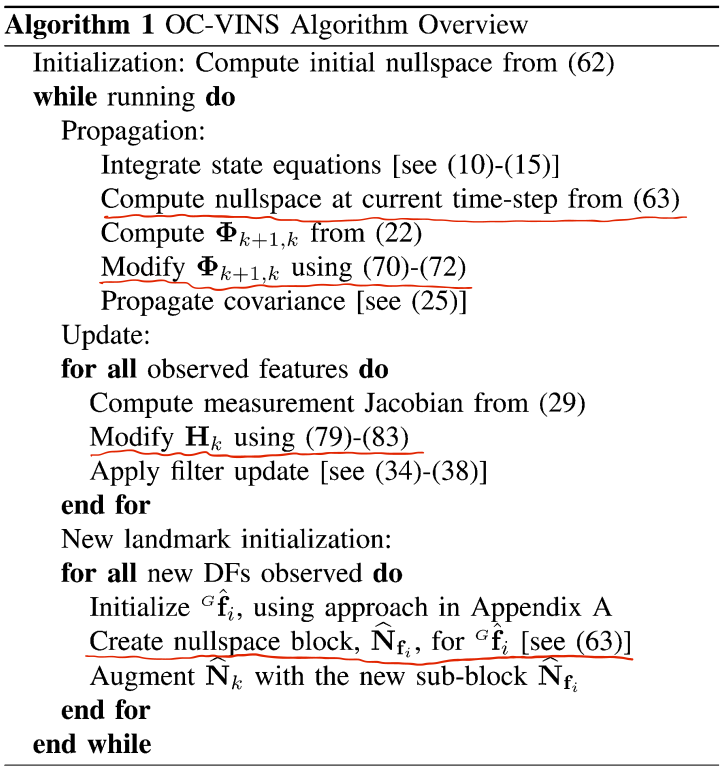#### App: OC-MSC-KF

MSCKF-VIO (S-MSCKF):

Modification of the State Transition Matrix $$\Phi$$

Modification of the Measurement Jacobian $$H$$

# Gauge Freedom Handling

It is well known that visual-inertial systems have four degrees offreedom that are not observable: the global position and the rotationaround gravity. These unobservable degrees of freedom (called gaugefreedom) have to be handled properly in visual-inertial state estimationto obtain a unique state estimate.

H有正确的零空间，比如，对于单目VO，rank(N(H)) =7，则H为奇异矩阵，那么增量方程始终存在病态或求解不稳定问题；通过处理规范自由度 解决。

In optimization-based methods, three approaches are usually used:

• Gauge Fixation: fixing the initial state,
• Gauge Prior: adding a prior to the initialstate,
• Free Gauge: allowing the parameters to evolvefreely during optimization.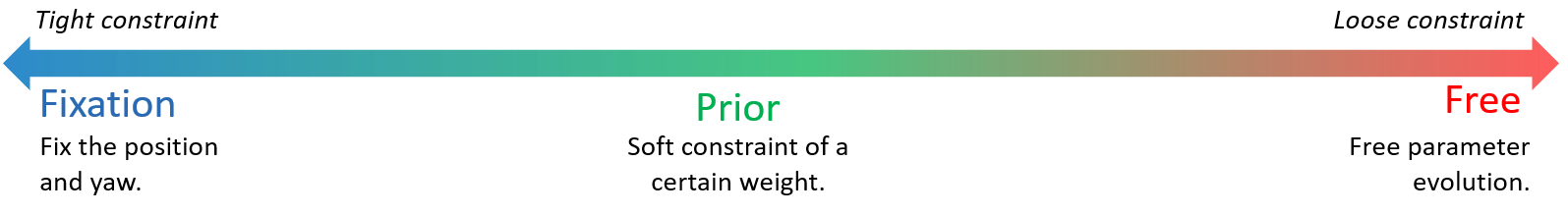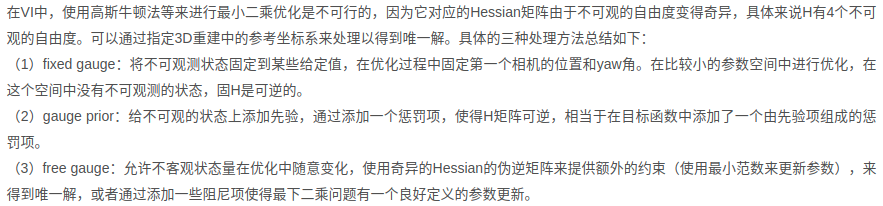ref:

# FAQ

• 为什么位置不可观，对于单目VO，第一帧不是固定住了吗？

# Reference

]]>
<p>[TOC]</p> <h1 id="overview">Overview</h1> <p>What is <strong>observability</strong> ?</p> <blockquote> <p>In control theory, observabilit
PyTorch on Ubuntu 18.04 https://cgabc.xyz/posts/70f98416/ 2022-06-24T16:00:00.000Z 2022-08-26T12:03:32.804Z [TOC]

# Install PyTorch locally

• https://pytorch.org/get-started/locally/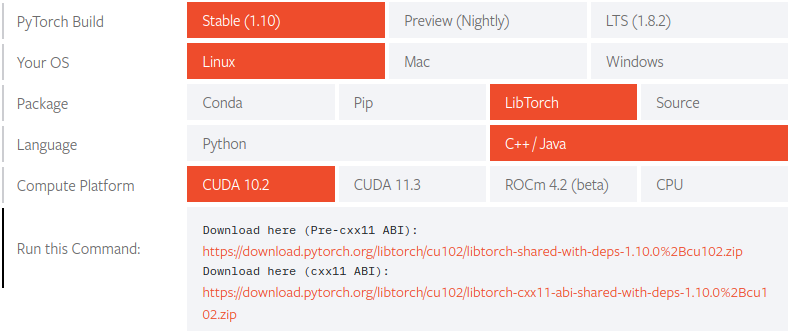test

## LibTorch (C++)

• Build from Source

The built libtorch library is located atpytorch/torch/lib/tmp_install/ in default.

• use with cmake

• include dir

# PyTorch 模型文件

• PyTorch的模型文件一般会保存为 .pth文件，C++接口一般读取的是 .pt 文件
• .pth 文件通过 torch.jit.trace转换后得到 .pt 文件

# FAQ

UserWarning: CUDA initialization: CUDA unknown error - this may bedue to an incorrectly set up environment, e.g. changing env variableCUDA_VISIBLE_DEVICES after program start. Setting the available devicesto be zero

]]>
<p>[TOC]</p> <h1 id="install-pytorch-locally">Install PyTorch locally</h1> <ul> <li>https://pytorch.org/get-started/locally/</li> </ul> <p a
Yolo Test on Ubuntu 18.04 https://cgabc.xyz/posts/65ad6508/ 2022-06-24T16:00:00.000Z 2022-08-26T12:03:32.804Z [TOC]

# YOLO

• Darknet: Open SourceNeural Networks in C

• YOLO: Real-Time Object Detection

## Detection Using APre-Trained Model• output

• fixed CUDA Error with the cfg code below

out of memory

# Yolo for ROS

• https://github.com/leggedrobotics/darknet_ros

• YOLO ROS: Real-Time Object Detection for ROS

## cfg files

• darknet_ros.launch

• ros.yaml

## Build

]]>
<p>[TOC]</p> <h1 id="yolo">YOLO</h1> <ul> <li><p><a href="https://pjreddie.com/darknet/">Darknet</a>: Open Source Neural Networks in C</p></
Image Exposure Controller https://cgabc.xyz/posts/7d4dd820/ 2022-06-21T16:00:00.000Z 2022-08-26T12:03:32.800Z
• msckf-vio Issue: About image exposure controller #41

• The auto exposure code is not open source because for one the actualimplementation is ugly, and second because it ties into other softwarethat is not open sourced.

Here a few (edited) notes that I sent to somebody who asked about howit works:

• what we implemented is super simple but works well inpractice:

$\text{new-shutter-time} = \text{current-shutter-time} \cdot\frac{\text{desired-brightness}}{\text{current-brightness}}$

You can go fancier by implementing

$\text{new-shutter-time} = \text{current-shutter-time} \cdot\left(\frac{\text{desired-brightness}}{\text{current-brightness}}\right)^\alpha$

$$\alpha \leq 1$$, but in practice$$\alpha = 1$$ works justfine.

• brightness is computed from every 16throw and column, so in fact only 1 out of every 256 is used forbrightness computation

• threshold on brightness:

we only change shutter time if

$|\text{desired-brightness} - \text{current-brightness}| >\text{threshold}$

This is so that we don't torture the ptgrey cameras with constantshutter speed changes.

• region of interest (ROI) support: we compute brighnessonly from the bottom X%. Typically X=70, so wewill ignore the top 30% of the image, because that's often where the sunor sky are, and where we don't usually pick up features.

• configurable max shutter limit: the max shuttertime is the min of the shutter time imposed by frame rate, and aconfigurable hard shutter limit (in case we get motion blur and decidewe'd rather live with a darker image and/or gain noise than themotion blur).

• auto gain: once we hit max shutter, we turn onauto gain (and when it get's brighter again, we take the gain off first,then decrease shutter).

So

$\text{if} \; \left| \bar{B} - B_c \right| > B_{th}: \\ \; T_s\leftarrow T_s \cdot \frac{\bar{B}}{B_c} \\ \; \text{if} \; T_s <T_{min}: \\ \text{auto gain}$

# Implementation

• image_exposure_control for RS cam

• vo-autoexpose: An auto-exposure algorithm for maxing out VO performance inchallenging light conditions

# Reference

]]>
<ul> <li>msckf-vio Issue: About image exposure controller #41 <sup id="fnref:1" class="footnote-ref"><a href="#fn:1" rel="footnote"><span cl
A-LOAM https://cgabc.xyz/posts/79cfdce/ 2022-06-18T16:00:00.000Z 2022-08-26T12:03:32.808Z [TOC]

# Overview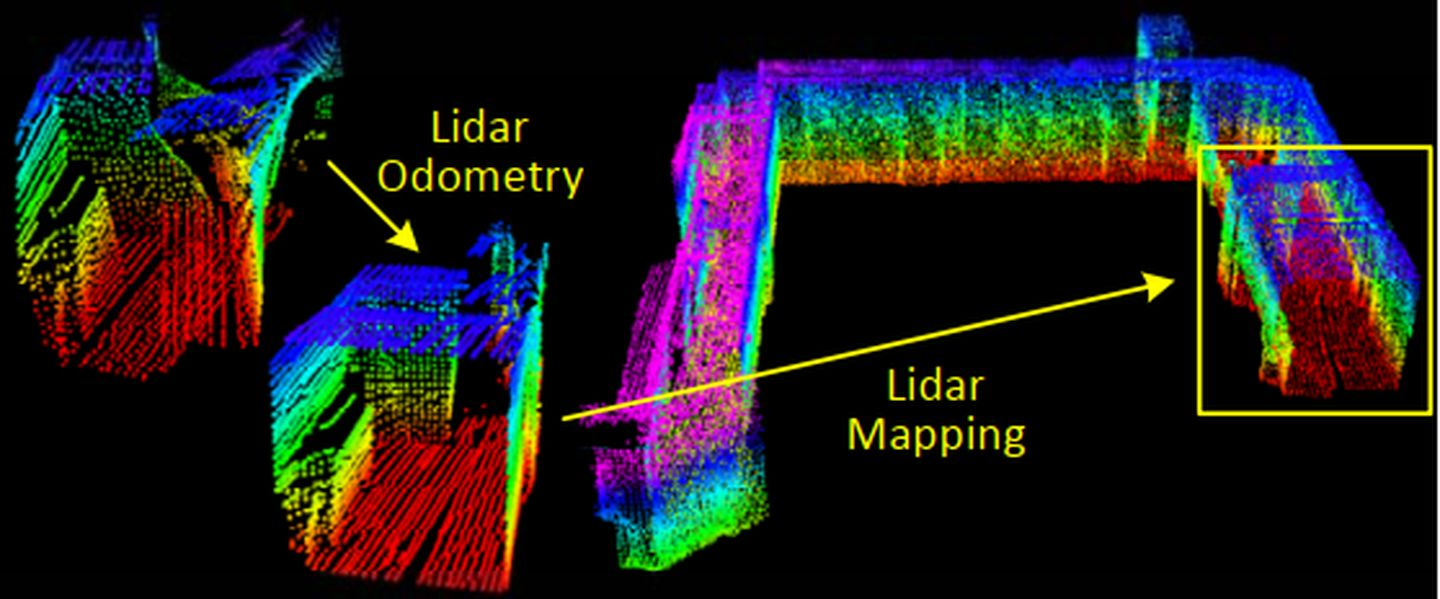A-LOAM is an Advanced implementation of LOAM (J. Zhang and S. Singh.LOAM: Lidar Odometry and Mapping in Real-time), whichuses Eigen and Ceres Solver to simplify code structure.

Code: A-LOAM 注释版

ROS Graph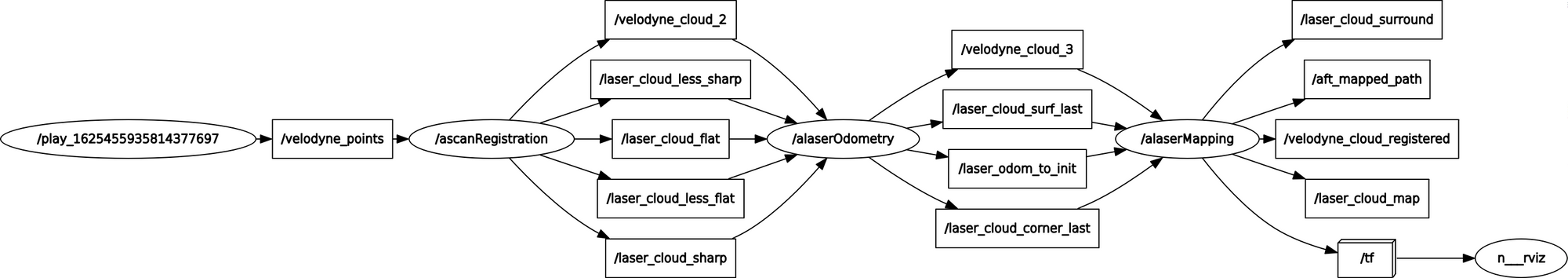Pipeline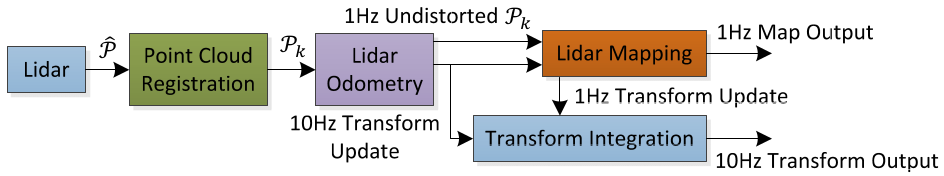# Lidar Hardware

## Hokuyo UTM-30LX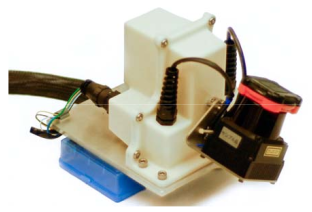• Vertical
• sweep: $$180^\circ /s$$, a rotation from $$-90^\circ$$ to $$90^\circ$$ or in the inverse direction(lasting for 1s)
• FOV: $$180^\circ$$
• scan rate: 40 lines/sec
• resolution: $$180^\circ /40 = 4.5^\circ$$
• Horizontal (a scan plane)
• resolution: $$0.25^\circ$$ within a scan
• angular speed: $$180^\circ$$ between $$-90^\circ$$ and $$90^\circ$$ with the horizontal orientationof the laser scanner as zero

## VLP-16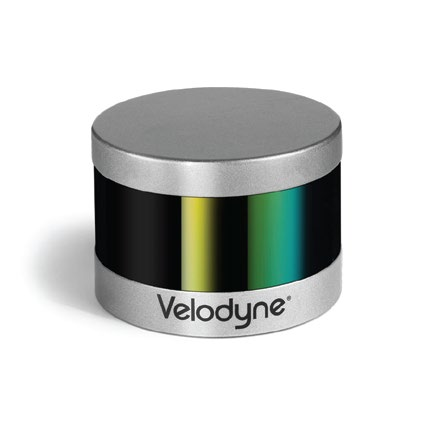• Time of flight distance measurement with calibratedreflectivities
• 16 channels
• Measurement range up to 100 meters
• Accuracy: +/- 3 cm (typical)
• Dual returns
• Field of view (vertical): 30° (+15° to -15°)
• Angular resolution (vertical): 2°
• Field of view (horizontal/azimuth): 360°
• Angular resolution (horizontal/azimuth): 0.1° -0.4°
• Rotation rate: 5 - 20 Hz

# Scan Registration

## 数据预处理

• 数据清洗

• 按线数保存的点云集合

• 曲率计算 (使用每个点的前后五个点)

## 特征提取

根据曲率进行点云特征提取，将每条线上的点分入相应的类别：边沿点和平面点

• sharp edges
• planar surface patches

对于每条线

• 将每个scan的曲率点分成6等份处理,确保周围都有点被选作特征点

对于每一份，曲率大于0.1的点

• 挑选曲率最大的前2个点放入sharp点集合cornerPointsSharp，同时cloudLabel[ind] = 2
• 挑选曲率最大的前20个点放入less sharp点集合cornerPointsLessSharp，同时cloudLabel[ind] = 1
• 点的前后各5个连续距离比较近的点筛选出去，防止特征点聚集，使得特征点在每个方向上尽量分布均匀

对于每一份，曲率小于0.1的点

• 放入flat点集合 surfPointsFlat，同时cloudLabel[ind] = -1
• 点的前后各5个连续距离比较近的点筛选出去，防止特征点聚集，使得特征点在每个方向上尽量分布均匀

对于每一份，将剩余的点cloudLabel[k] <= 0（包括之前被排除的点）全部归入平面点surfPointsLessFlatScan

# Odometry（高频率，粗定位）

## 运动畸变矫正

运动畸变示意图如下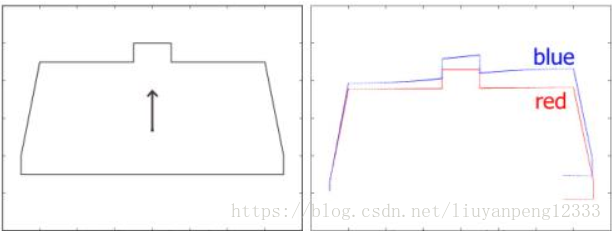Reprojecting point cloud to the end of a sweep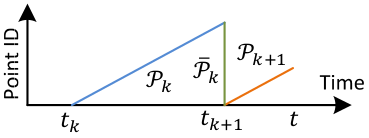## 特征匹配 (Scan-Scan)

### correspondence for cornerfeatures

当前点 curr_point线段匹配，找到线段的两个端点

• last_point_a: KDTree 搜索最近的点
• last_point_b:在scan增长和下降的方向上分别搜索，不在同一scan但处于一定阈值scan范围内，距离最小的点

### correspondence for planefeatures

当前点 curr_point匹配，找到面的三个点

• last_point_a: KDTree 搜索最近的点

• last_point_b:在scan增长(intensity<=closestPointScanID)和下降(intensity>=closestPointScanID)的方向上分别搜索，处于一定阈值scan范围内，距离最小的点

• last_point_c:在scan增长(intensity>closestPointScanID)和下降(intensity<closestPointScanID)的方向上分别搜索，处于一定阈值scan范围内，距离最小的点

残差度量方式

• 点到线段距离
• 点到面距离

# Mapping（低频率，精定位）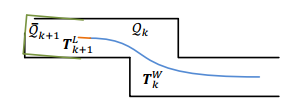## 基于Cube的地图管理

LOAM采用的是栅格（cube）地图的方法，将整个地图分成21×21×11个珊格，每个珊格是⼀个边⻓50m的正⽅体，当地图逐渐累加时，珊格之外的部分就被舍弃，这样可以保证内存空间不会随着程序的运⾏⽽爆掉，同时保证效率。

## 特征匹配 (Scan-Map)

将当前帧已经消除畸变的点云转换到全局坐标系transformAssociateToMap()，然后与局部地图（localmap或者称为submap，源码中使用的是三维栅格cube做的局部地图管理）做特征匹配

用于特征匹配的局部地图 (local map)

### correspondence for cornerfeatures

当前点 curr_point线段匹配，找到线段的两个端点

KDTree 搜索最近的5个点（最远点距离小于1米），计算其中心点center，并构建协方差矩阵；如果是线特征，协方差矩阵最大特征值对应的特征向量即为线的方向向量unit_direction，然后根据中心点和方向向量得到两个端点

• last_point_a
• last_point_b

### correspondence for planefeatures

当前点 curr_point匹配，找到面的法向量

KDTree 搜索最近的5个点（最远点距离小于1米），计算面的法向量

## 运动估计 ICP

残差度量方式

• 点到线段距离
• 点到面距离

计算出的位姿修正Odometry的位姿

## 地图增长

获得 laserCloudCornerArraylaserCloudSurfArray，并降采样；当地图逐渐累加时，珊格之外的部分就被舍弃，这样可以保证内存空间不会随着程序的运⾏⽽爆掉，同时保证效率。

]]>
<p>[TOC]</p> <h1 id="overview">Overview</h1> <p align="center"> <img src="/img/post/loam/loam_pc.png" style="width:80%"> </p> <p>A-LOAM is a
OpenMVG + OpenMVS build & run https://cgabc.xyz/posts/13a6e139/ 2022-06-17T16:00:00.000Z 2022-08-26T12:03:32.800Z [TOC]

# Overview

• OpenMVG providesan end-to-end 3D reconstruction from images framework compounded oflibraries, binaries, and pipelines.

• a library mainly focused on Multiple-View-Geometryand Structure-From-Motion
• Structure-from-Motion pipelines (likeOpenMVG) which recover camera poses and a sparse 3Dpoint-cloud from an input set of images, there are none addressing thelast part of the photogrammetry chain-flow• OpenMVS(Multi-View Stereo) is a library for computer-vision scientists andespecially targeted to the Multi-View Stereoreconstruction community.

• Modules

• aims at filling that gap by providing a complete set ofalgorithms to recover the full surface of the scene to bereconstructed

• The input is a set of camera poses plus the sparse point-cloudand the output is a textured mesh

# OpenMVG

## Build

• https://github.com/openMVG/openMVG/blob/develop/BUILD.md

## Run

Modify the code below in SfM_SequentialPipeline.py,SfM_GlobalPipeline.py ortutorial_demo.py

then

MVG (SfM scene) to MVS

# OpenMVS

## Build

• https://github.com/cdcseacave/openMVS/wiki/Building

## Run

### View

Viewer module can be used to visualize anyMVS project file or PLY/OBJ file.

# Ref

]]>
<p>[TOC]</p> <h1 id="overview">Overview</h1> <ul> <li><p><a href="https://github.com/openMVG/openMVG">OpenMVG</a> provides an end-to-end 3D
Mesh Texturing in a Nutshell (Let There Be Color) https://cgabc.xyz/posts/d001e9db/ 2022-06-11T16:00:00.000Z 2022-08-26T12:03:32.800Z [TOC]

# Overview

digraph { TV [label="TextureView"]; TVs [label="N TextureViews"];Texturing [style=filled, shape=box]; ColorImg->TV; CamK->TV;CamTF->TV; TV->TVs; TVs->Input; TriangleMesh->Input;Input->Texturing->Output->TexturedMesh; }
• code (forked): https://github.com/cggos/mvs-texturing
• paper: Let There Be Color! Large-Scale Texturing of 3DReconstructions

# 1. Texture Views

digraph { TV [label="TextureView"]; TV->ColorImg; TV->CamK;TV->CamTF; }

# 2. Mesh --> MeshInfo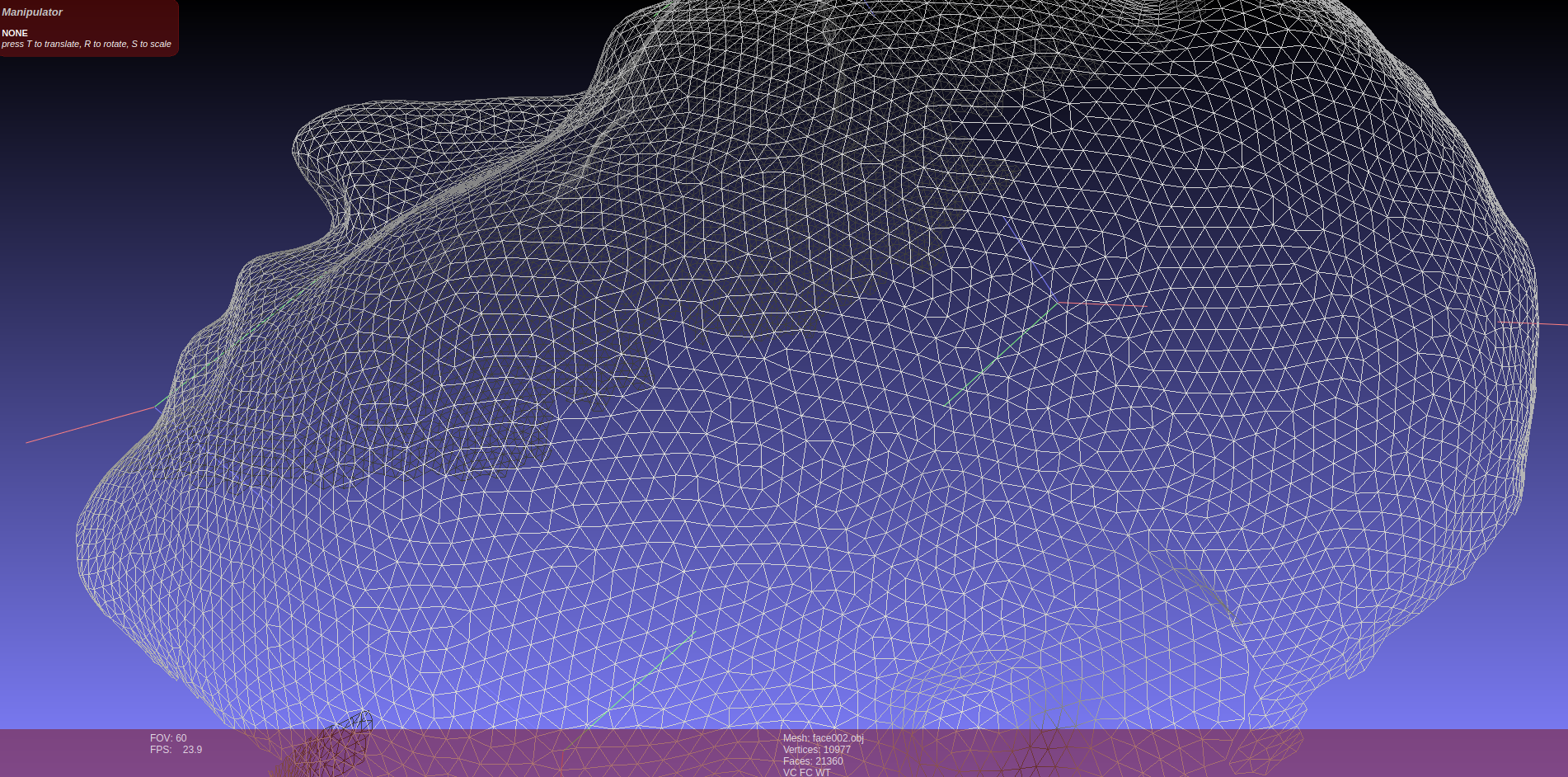## Check Mesh

• Ensure face and vertex normals

## Init MeshInfo

### Create VertexInfo

Add faces to their three vertices

digraph { vertex -> face1 vertex -> face2 vertex -> face3 }

### Update VertexInfo

Classify each vertex and compute adjacenty info

• Build new, temporary adjacent faces representationAdjacentFaceList adj_temp for ordering

digraph { face_id [color=green]; front_vid [color=blue]; back_vid[color=blue];

graph { layout=twopi; node [shape=circle];

v0 [color="red"]; v1 [color="blue"]; v2 [color="blue"];

v0--v1 [color=green]; v0--v2 [color=green]; v0--v3; v0--v4; v1--v2[color=green]; v3--v2; v3--v4; v1--v4;

overlap=false; }
• Sort adjacent faces by chaining them

• update VertexInfo

digraph { vclass; verts [color=blue]; faces [color=green];

vinfo->vclass; vinfo->verts; vinfo->faces; }

# 3. Mesh + MeshInfo--> Adjacency Graph (UniGraph)

对于每个 face，将mesh中与其每条 edge 邻接的 face 存入adj_faces；将当前 face 与 adj_faces 中每个face 建立 edge，构建 UniGraph

graph { node [shape=circle];

f0--f1; f1--f2; f0--f3; f3--f4; f1--f4;

overlap=false; }

# 4. View Selection--> Best View Label 😄## Calculate DataCosts

Calculates the data costs for each face and texture view combination,if the face is visible within the texture view.

### CalculateFaceProjectionInfo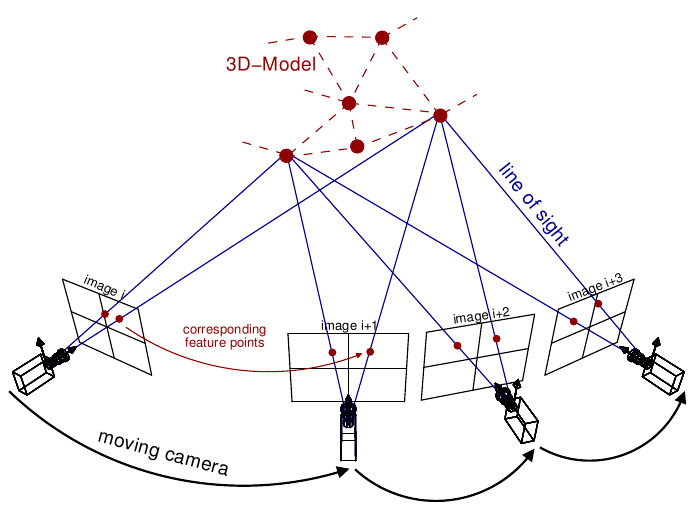digraph { face_info [style=filled]; nnn [label="..."]; face_info_n[style=filled]; rankdir=LR; face_id->face_info;face_info->view_id; face_info->mean_color; face_info->quality;face_id->nnn; face_id->face_info_n; }

### PostProcess Face Infos

create hist_qualities::Histogram usinginfo.quality, and get the upper_bound whenpercentile=0.995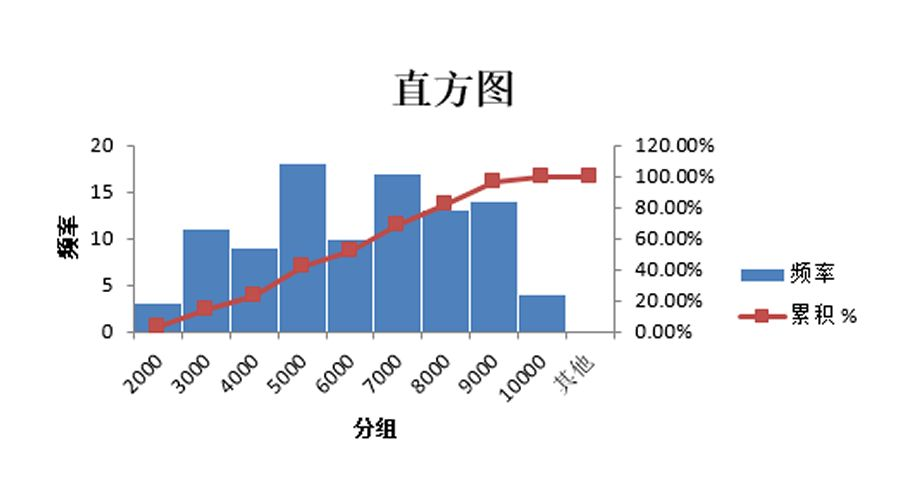compute data cost

• gmi
• area
DataCostface0face1...faceN
view0
view1
...
viewN

## View Selection

### Data Association

#### Graphmapmap::Graph<cost_t>

graph { rankdir = LR; face_id--adj_face_id [label="weight"]; }

#### LabelSetmapmap::LabelSet<cost_t, simd_w>

view idface0face1...faceN
view0
view1
...
viewN

#### Unaries

face_idlabel_setcosts
unary0
unary1
...
unaryN

### MAP-MRF🚩The aim is to find a labeling for X that producesthe lowest energy.

#### pairwise MRFs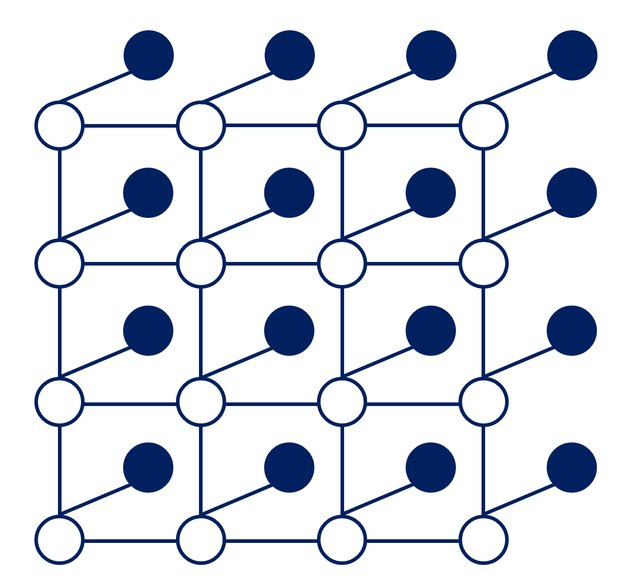• the filled-in circles: the observed nodes $$Y_i$$ (face)
• the empty circles: the "hidden" nodes $$X_i$$ (view label)

#### MAP --> Minimum Energy

energy/cost function:

$\text{energy} (Y, X) =\sum_{i} \text{DataCost} (y_i, x_i) +\sum_{j = \text{neighbours of i}} \text{SmoothnessCost} (x_i, x_j)$

##### Tree MRFs via DP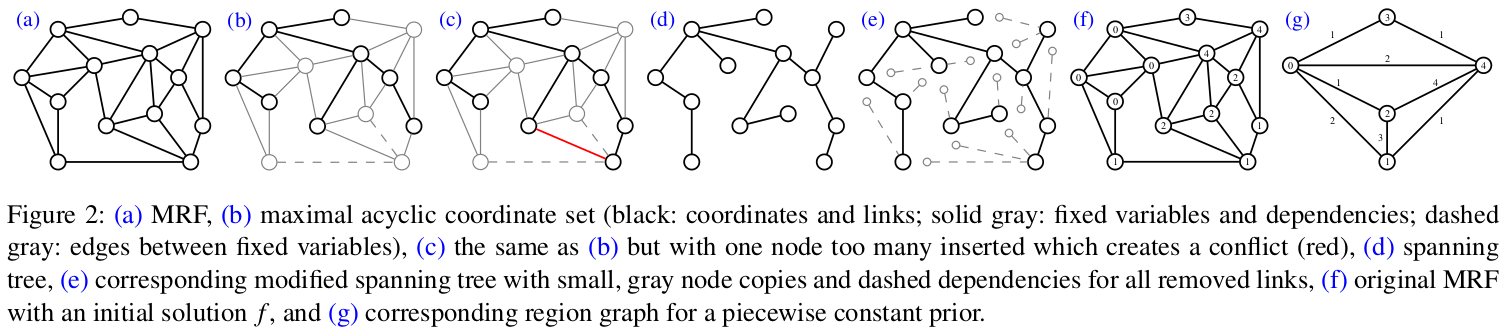##### LBP

by OpenMVS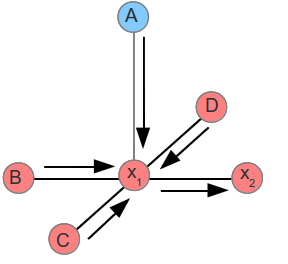# 5. Create Texture Atlases😄## Generate Texture Patches

Generates texture patches using the graph to determine adjacent faceswith the same label.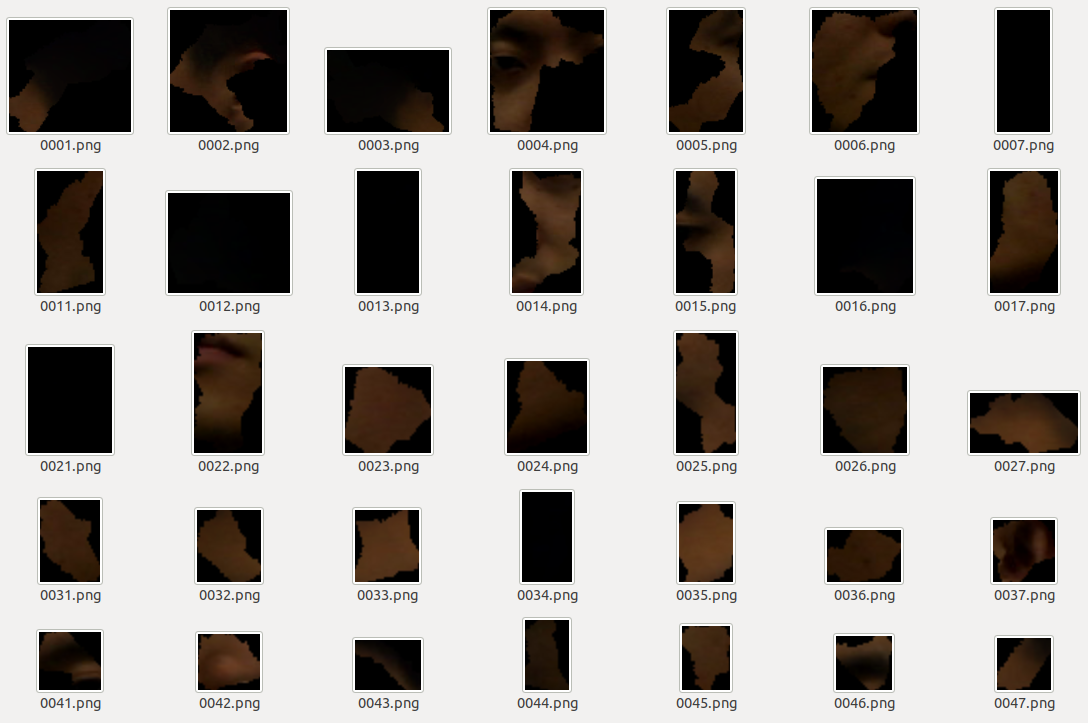## Global /Local Seam Levelling 🚩• paper: Seamless Mosaicing of Image-Based Texture Maps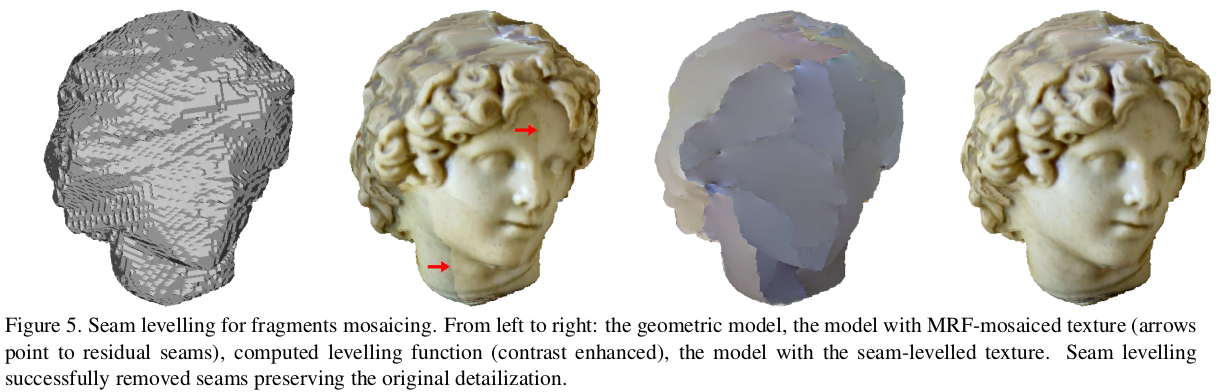without seam levelling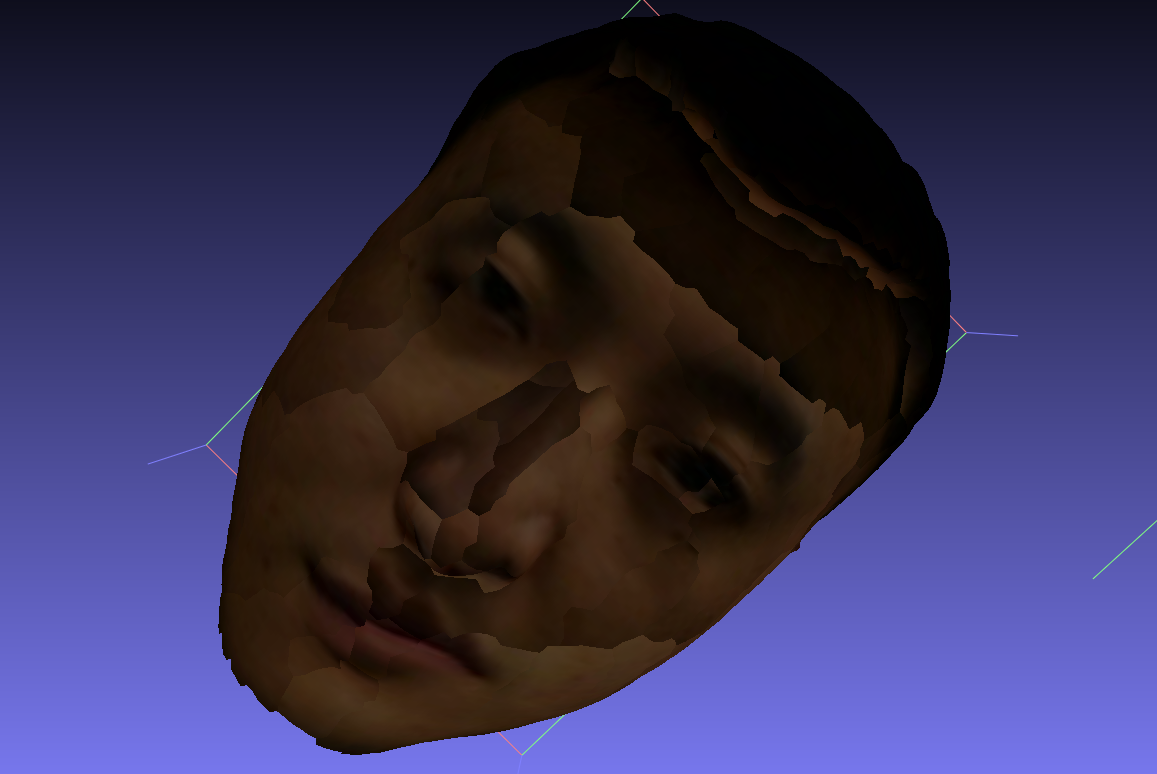## Texture Atlases

generate TextureAtlas from all ofTexturePatch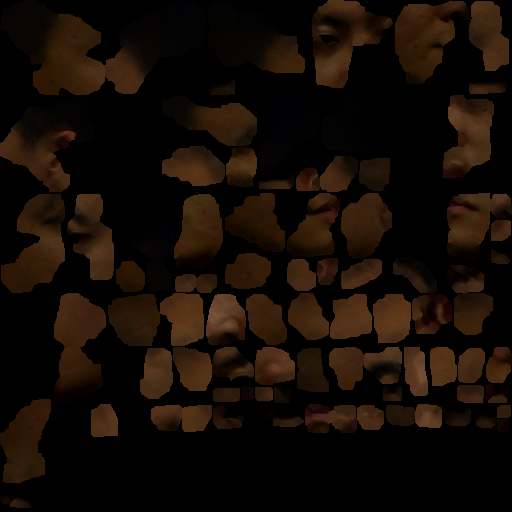# 6. Mesh + Texture --> ObjModel

• .obj
• .mtl
• .png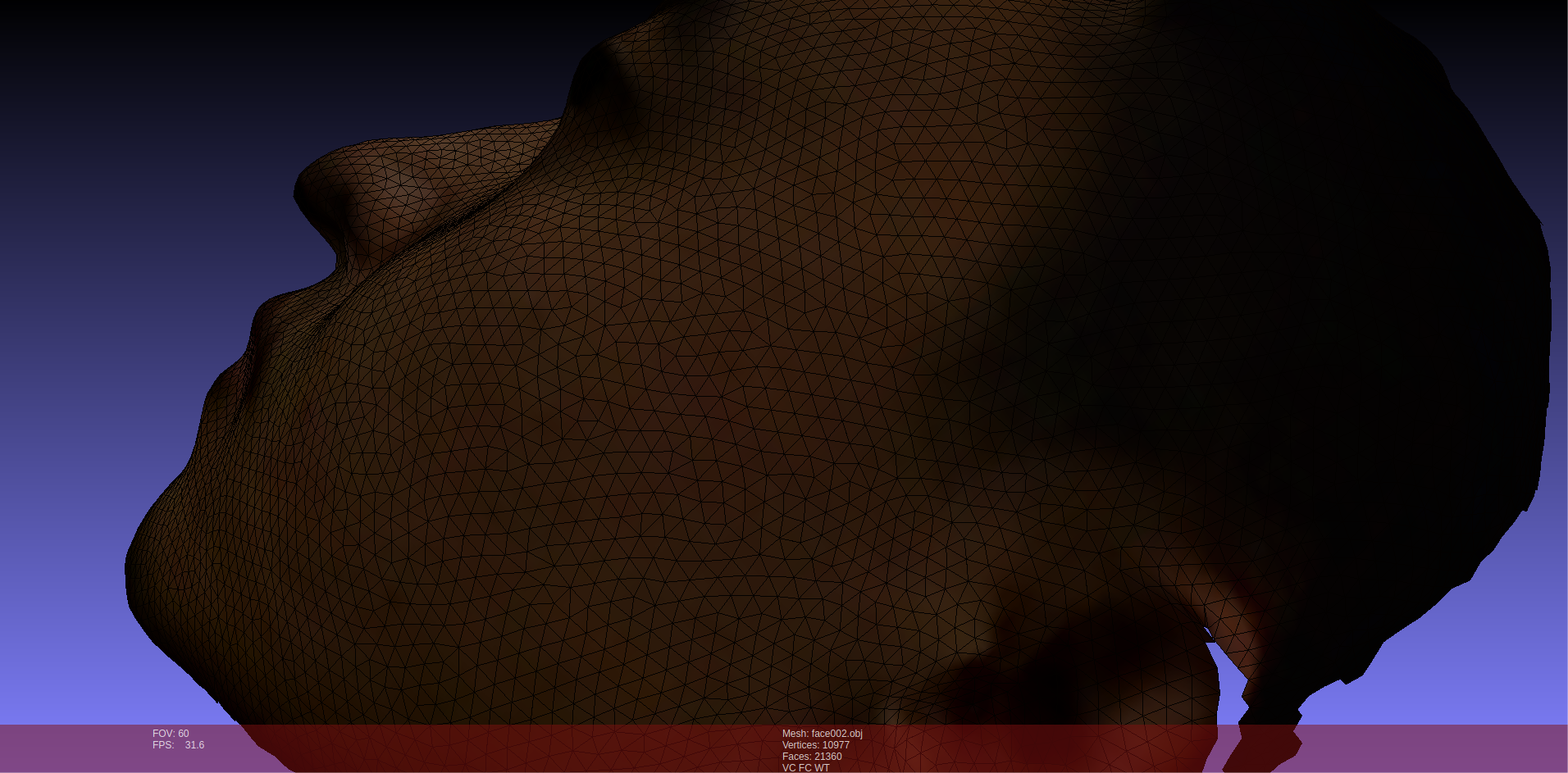# 网格UV展开

上述纹理重建属于 计算机视觉的内容，本节是其逆过程，属于 计算机图形学 的内容。

• http://geometryhub.net/notes/uvunfold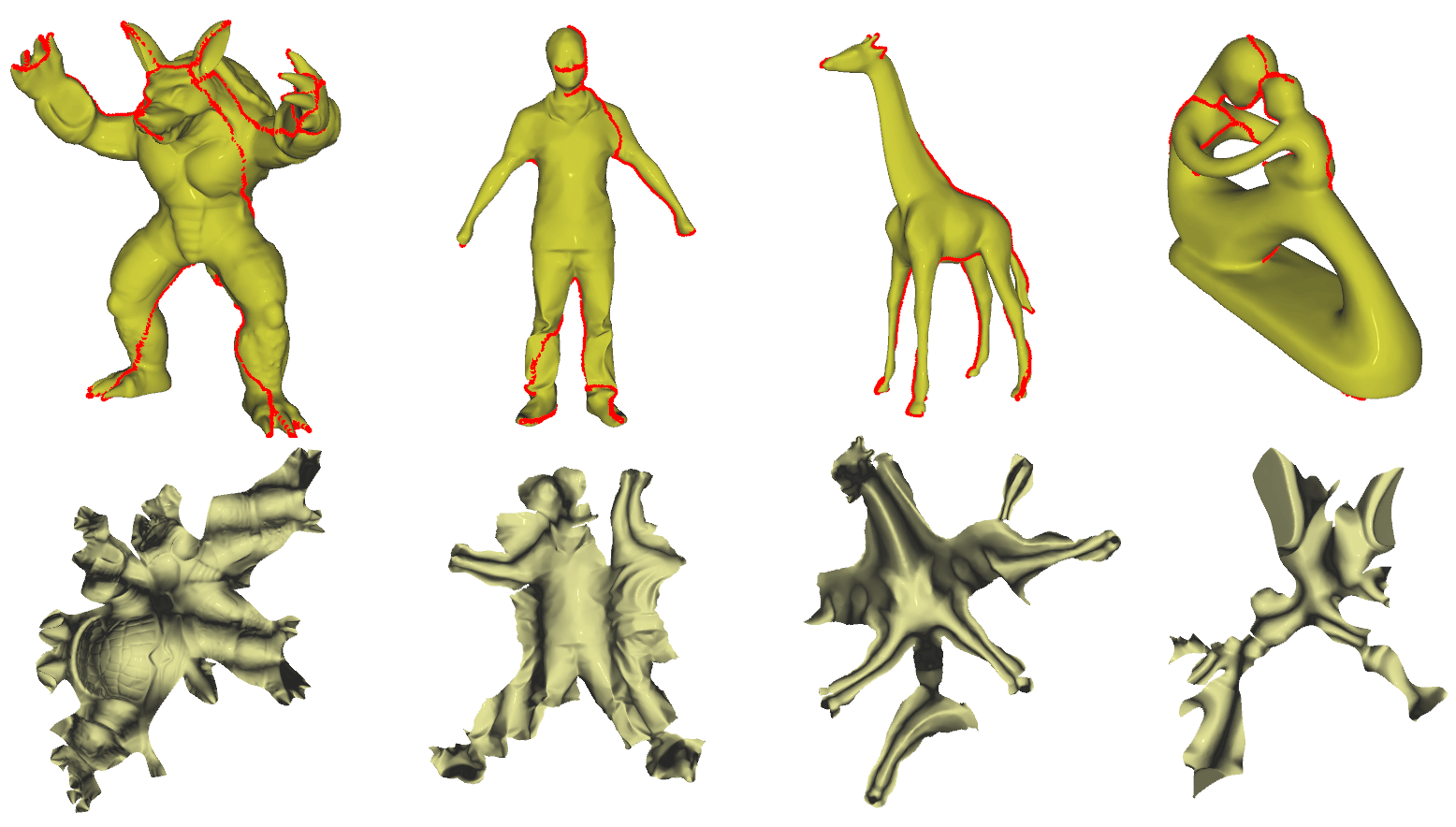# Reference

]]>
<p>[TOC]</p> <style> .graphviz { display: flex; justify-content: center; } </style> <h1 id="overview">Overview</h1> <!-- dot {align="
Kinect Fusion https://cgabc.xyz/posts/c93e15aa/ 2022-06-09T16:00:00.000Z 2022-12-24T07:17:24.344Z [TOC]

# Overview

Kinect Fusion 描述三维空间的方式叫Volumetric。它把固定大小的一个空间（比如3𝑚×3𝑚×3𝑚）均匀分割成一个个小方块（比如512×512×512），每个小方块就是一个voxel，存储TSDF值以及权重。最终得到的三维重建就是对这些voxel进行线性插值。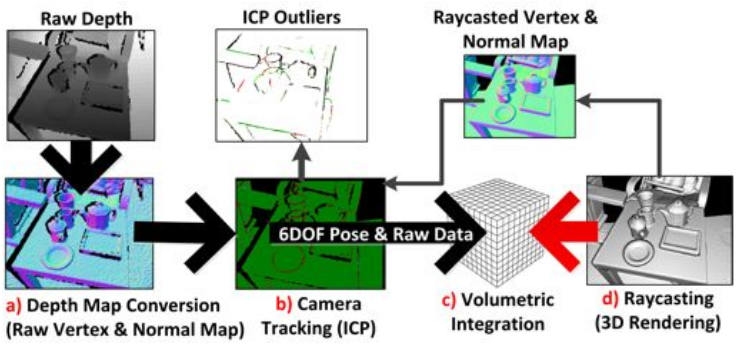重建流程如上图所示：

• Depth Map Conversion:读入的深度图像转换为三维点云并且计算每一点的法向量

• Camera Tracking (map-to-frame ICP):计算得到的带有法向量的点云，和通过光线投影算法根据上一帧位姿从模型投影出来的点云，利用ICP 算法配准计算位姿

• Volumetric Integration:根据计算得到的位姿，将当前帧的点云融合到网格模型中去，这里用到了TSDF

• Raycasting (光线投影算法):根据当前帧相机位姿利用该算法从模型投影得到当前帧视角下的点云，并且计算其法向量，用来对下一帧的输入图像配准

如此是个循环的过程，通过移动相机获取场景不同视角下的点云，重建完整的场景表面。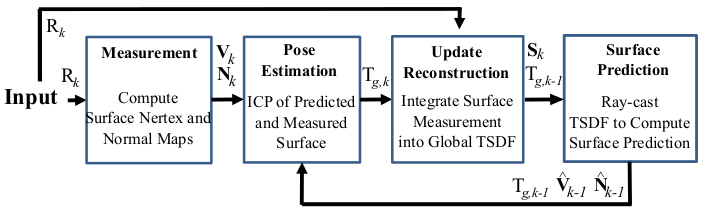Project:

# Depth Map Conversion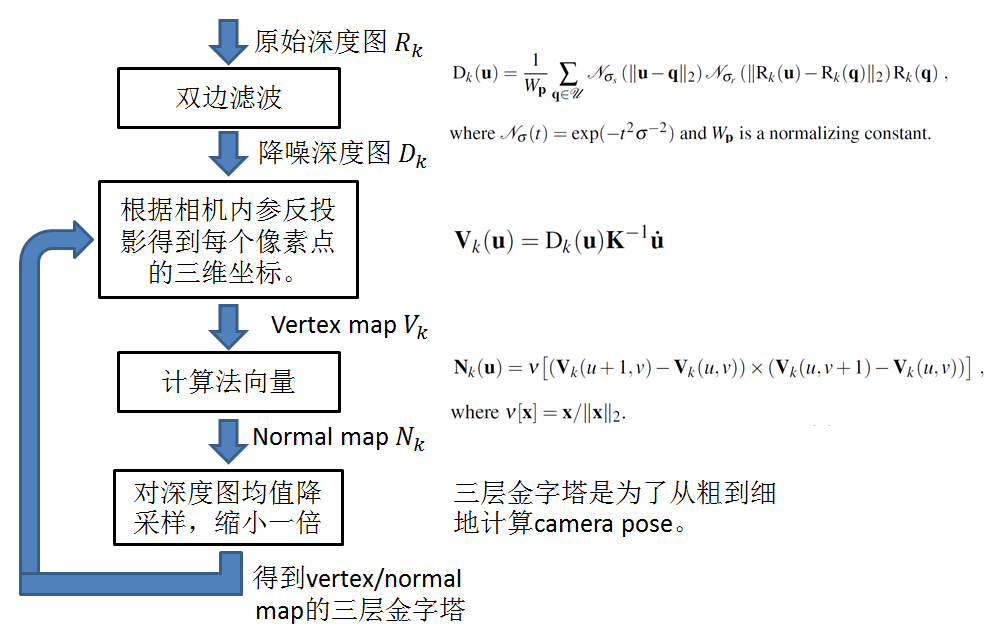构建三层金字塔的目的是为了从粗到细地计算相机位置姿态，有加速计算的效果。

# Camera Tracking (ICP)

相机的位置姿态是用ICP (Iterative Closest Point)求解的。ICP是处理点云的常规手段，通过最小化两块点云的差别，迭代求解出拍摄两块点云的相机之间的相对位置。

有不同的方式来描述点云的差别，最常用的是point-to-point和point-to-plane两种。KinectFusion选择的是point-to-plane的方式，要把点到点的距离向法向量投影。point-to-plane要比point-to-point收敛速度快很多，而且更鲁棒。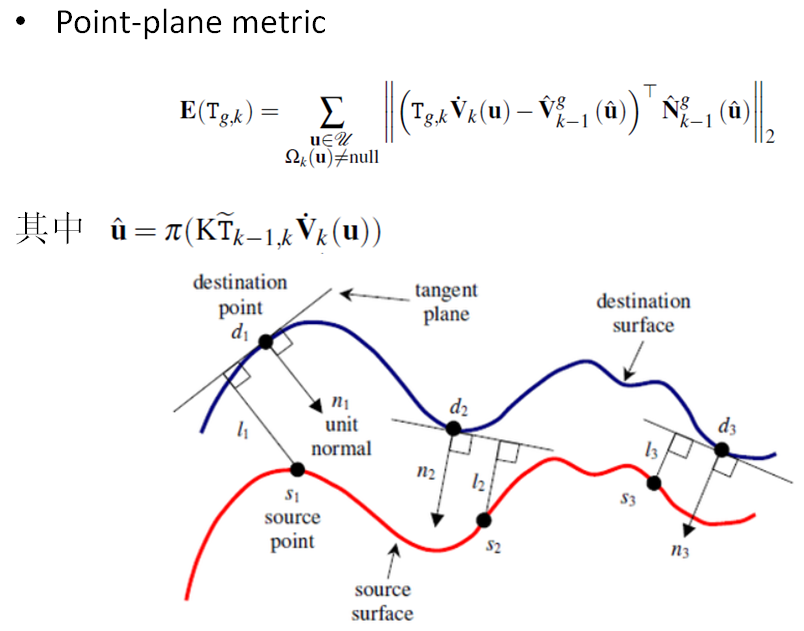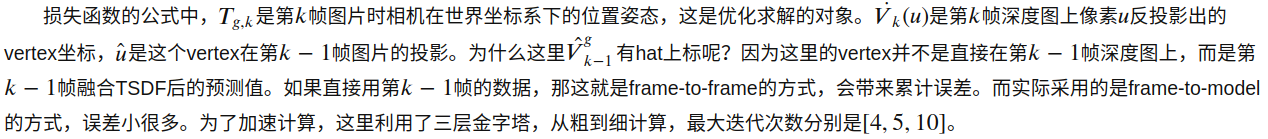KinectFusion 算法采用 frame-to-model（通过当前帧深度图像转换得到的点云，和根据上一帧相机位姿从模型投影获取的深度图像转换得到的点云进行配准）的方式，而不是采用frame-to-frame（通过当前帧深度图像转换得到的点云，和上一帧深度图像转换得到的点云进行配准）的形式计算两帧位姿，作者论文里也验证了采用frame-to-model 的形式重建要更加准确。

假设poseestimation已经计算出来，就可以把本次测量结果融合到全局地图（globalmodel）中了。

这里的model使用的是TSDF地图

# Volumetric Integration (TSDF)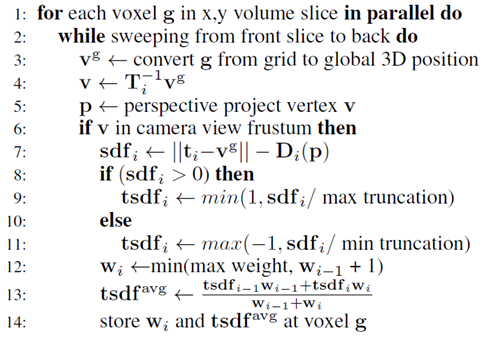# Surface Reconstruction(Raycast TSDF)

更新完TSDF值之后，就可以用TSDF来估计 voxel/normalmap。这样估计出来的voxel/normalmap比直接用RGBD相机得到的深度图有更少的噪音，更少的孔洞（RGBD相机会有一些无效的数据，点云上表现出来的就是黑色的孔洞）。估计出的voxel/normalmap与新一帧的测量值一起可以估算相机的位置姿态。

## Ray-Casting

具体的表面估计方法叫Raycasting。这种方法模拟观测位置有一个相机，从每个像素按内参𝐾投射出一条射线，射线穿过一个个voxel，在射线击中表面时，必然穿过TSDF值为一正一负的两个紧邻的voxel（因为射线和表面的交点的TSDF值为0），表面就夹在这两个voxel里面。然后可以利用线性插值，根据两个voxel的位置和TSDF值求出精确的交点位置。这些交点的集合就呈现出三维模型的表面。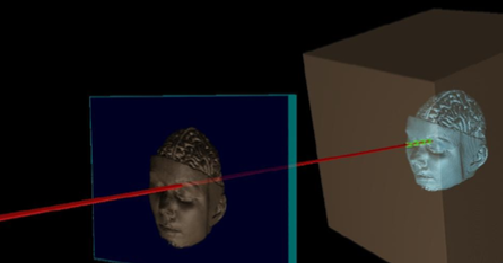如图：从光心出发，穿过像素点在网格模型中从正到负的穿越点，就表示在当前像素点处可以看到的重建好的场景的表面。对于每个像素点，分别做类似的投影，就可以计算得到的在每个像素点处的点云。

采用光线投影算法计算得到的点云，再计算其法向量，用带法向量的点云和下一帧的输入图像配准，计算下一帧输入图像的位姿。如此是个循环的过程。

其实，到此KinectFusion的流程已经结束了，重建出了点云格式的表面三维模型；但在实际应用中，尤其AR领域，还需要Mesh格式的三维模型，甚至需要纹理贴图等。

# Mesh Generation

## Marching Cube

通过Marching Cube对重建后的点云实现三角面片重建。

点云数据在三维空间中为离散表示，对TSDF地图使用MarchingCube算法来对等值面进行提取，实现三角面片重建。

MarchingCube算法基本思想是逐个处理标量场中的体素，分离出与等值面相交的体素，采用插值计算出等值面与立方体边的交点。根据立方体每一顶点与等值面的相对位置，将等值面与立方体边的交点按一定方式连接生成等值面，作为等值面在该立方体内的一个逼近表示。

MarchingCube用来提取TSDF体素中隐含存储的三维网格模型，实际上是提取TSDF中的0等值曲面。首先，遍历操作，通过遍历TSDF网格，定位并记录下与0等值曲面相交的体素点；然后，提取操作，对于前面记录下来的体素点，利用预存的网格索引及线性插值方法，提取出三角形面片网格，得到重建的三维几何模型。

# Texturing

上面 KinectFusion 的几个步骤属于 几何重建的过程，而在实际中，尤其AR领域，还要给 三维模型 进行 纹理重建(纹理贴图)

# Other OS Code

]]>
<p>[TOC]</p> <h1 id="overview">Overview</h1> <p>Kinect Fusion 描述三维空间的方式叫 <strong>Volumetric</strong>。它把固定大小的一个空间（比如3𝑚×3𝑚×3𝑚）均匀分割成一个个小方块（比
CMake for Visual Studio https://cgabc.xyz/posts/449abec3/ 2022-05-31T16:00:00.000Z 2022-08-26T12:03:32.792Z [TOC]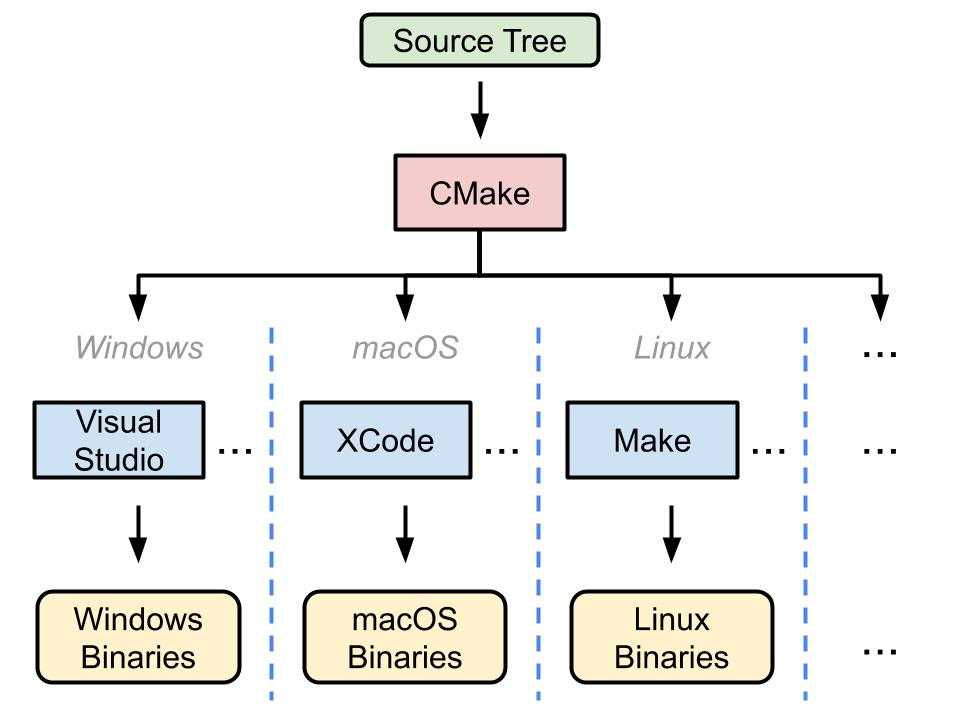# Property Sheet

example main.props, 在此文件设置工程，方便分享

example file

# Building System for VisualStudio

example Windows Batch file config.bat

# Build

## with cmake

from cmd with cmake --build

## with Visual Studio

打开 Visual Studio 工程 xxx.sln，生成解决方案

# Ref

]]>
<p>[TOC]</p> <p algin="cemter"> <img src="/img/post/cpp/cmake_general_diagram.jpg" style="width:60%"> </p> <h1 id="property-sheet">Property
求解方程数值近似解 https://cgabc.xyz/posts/52064f73/ 2022-04-30T16:00:00.000Z 2022-08-26T12:03:32.804Z Solve $$\sqrt{x}$$

### 基于泰勒公式的级数逼近

在 线性化点 $$x_0=1$$ 泰勒展开

$\sqrt{x} \cong 1+\frac{1}{2}(x-1)-\frac{1}{4} \frac{(x-1)^{2}}{2!}+\frac{3}{8} \frac{(x-1)^{3}}{3 !}-\frac{15}{16} \frac{(x-1)^{4}}{4!}+\cdots$

根据该公式我们可以在一定精度内逼近真实值，不过这个公式仍然存在一个问题，即是公式的收敛问题。

在泰勒级数展开中，平方根函数的公式当且仅当参数值位于一个有效范围内时才有效，在该范围内计算趋于收敛。该范围即是收敛半径，当我们对平方根函数用$$x_0=1$$进行计算时，泰勒级数公式希望x处于范围: $$0<x<2$$之间。如果x在收敛半径之外，则展开式中的项会越来越大，泰勒级数离答案也就越来越远。为了解决该问题，我们可以考虑当待开平方数大于4时以4去除它，最后将得到的数乘以相同次数的2即可。

# Reference

]]>
<h2 id="solve-sqrtx-12">Solve <span class="math inline">$$\sqrt{x}$$</span> <sup id="fnref:1" class="footnote-ref"><a href="#fn:1" rel="foot
DBoW Note https://cgabc.xyz/posts/edb02244/ 2022-03-24T16:00:00.000Z 2022-08-27T15:32:48.865Z [TOC]

# Overview

## Bag of Words

BoW（Bag ofWords，词袋模型），是自然语言处理领域经常使用的一个概念。一篇文章可能有一万个词，其中可能只有500个不同的单词，每个词出现的次数各不相同。词袋就像一个个袋子，每个袋子里装着同样的词。这构成了一种文本的表示方式。这种表示方式不考虑文法以及词的顺序。

## DBoW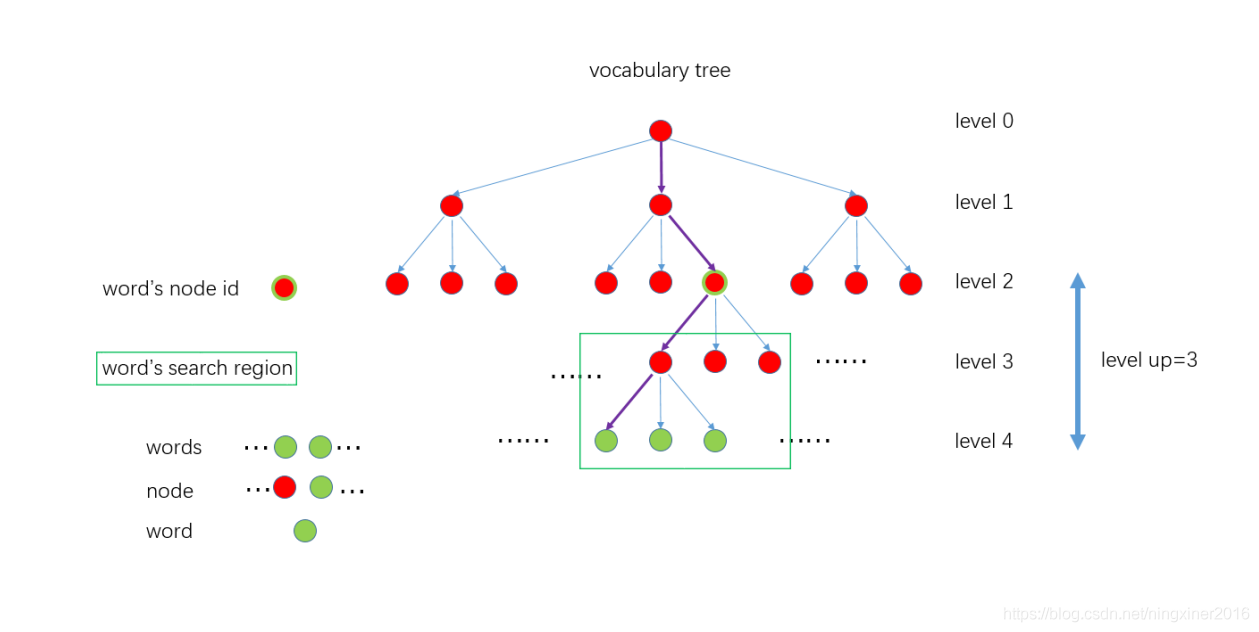在计算机视觉领域，图像通常以特征点及其特征描述来表达。如果把特征描述看做单词，那么就能构建出相应的词袋模型。这就是本文介绍的DBoW2库所做的工作。利用DBoW2库，图像可以方便地转化为一个低维的向量表示。比较两个图像的相似度也就转化为比较两个向量的相似度。它本质上是一个信息压缩的过程。

DBoW算法，来源于西班牙的Juan D. Tardos课题组，用于解决 PlaceRecognition问题，ORB-SLAM、VINS-Mono等SLAM系统中的闭环检测模块均采用了该算法，主要是基于词袋模型（BoW）https://en.wikipedia.org/wiki/Bag-of-words_model_in_computer_vision。

主要术语：

• 特征向量（FeatVec） - 单个视觉特征描述子
• 视觉单词 - 词典中的聚类中心，带有权重的单个视觉特征描述子
• 词袋向量（BowVec） - 一张图片用词袋中每个单词是否出现（+ 出现的次数+ TF-DF）组合而成的向量（体现多个视觉特征描述子）

主要过程：

• 构建字典（Vocabulary）：将图像数据库转换为索引图（k叉树）
• 近似最近邻（ANN）搜索：将一张图片中特征的描述子通过在k叉树种搜索转换为视觉单词（visualword），多个视觉单词组成词袋向量（BoW Vector）

# 离线步骤 -构建视觉字典（聚类问题，也称为无监督分类）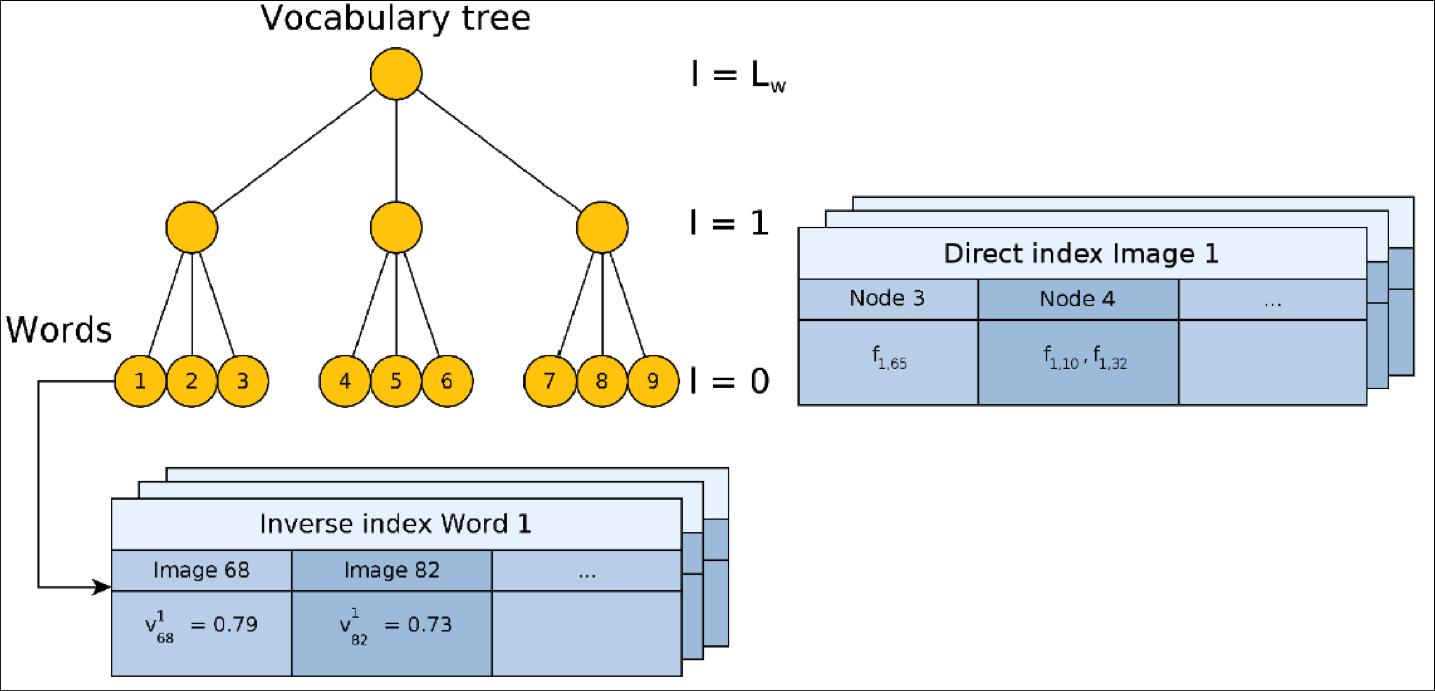主要采用K-means算法，将用于训练的图像数据库中的视觉特征（DBoW3中支持ORB和BRIEF两种二进制描述子）归入k个簇（cluster）中，每一个簇通过其质心（centroid）来描述，聚类的质量通常可以用同一个簇的误差平方和（Sumof SquaredError，SSE）来表示，SSE越小表示同一个簇的数据点越接近于其质心，聚类效果也越好。这里的“接近”是使用距离度量方法来实现的，不同的距离度量方法也会对聚类效果造成影响（后面会提到）。

将训练图像数据库中所有N个描述子分散在一个k分支，d深度的k叉树的叶子节点上，如分支数为3，深度为$$L_w$$，这样一个树结构有叶子结点 $$3^{L_w}$$个。可以根据场景大小、需要达到的效果修改k和d的数值。这样queryimage进来检索时，可以通过对数时间的复杂度（d次 = logkN）找到其对应的聚类中心，而不是使用O(n)的时间复杂度的暴力检索。据统计，在10000张trainimage图像数据库中找到queryimage的匹配图像耗时<39ms，并有较高的召回率和较低的falsepositive。

然后，为了提高检索时的效率、成功率以及准确率，还采用了下述权重计算算法

• 倒排索引（Inverse Index）
• 正排索引（Direct Index）
• TF-IDF（Term Frequency - Inverse DocumentFrequency）

K-means优点是容易实现，缺点是在大规模数据集上收敛较慢，并且可能收敛到局部最小，造成该簇没有代表性。对于描述子这种高维空间的大规模聚类，粗暴使用K-means会有问题。因此会使用其变种HierarchicalK-means 或 K-means++

## 实现过程

• 从训练图像中抽取特征
• 将抽取的特征用 k-means++算法聚类（使用汉明距离），将描述子空间划分成 k 类
• 将划分的每个子空间，继续利用 k-means++ 算法做聚类
• 重复$$L_w$$
• $$L_w$$次上述过程，将描述子建立成树形结构，如下图所示

k-means++代码在如下函数：

# 在线步骤 -近似最近邻检索（ANN Retrieval）

由于ORB和BRIEF描述子均为二进制，因此距离度量采用汉明距离（二进制异或计算）。queryimage的描述子通过在字典的树上检索（找到最近邻的叶子节点）视觉单词，组成一个词袋向量（BoWvector），然后进行词袋向量之间的相似度计算，得到可能匹配的rankingimages。最后还需要利用几何验证等方法选出正确（只是概率最大。。。）的那张图片。

## BoW2 in ORB-SLAM2

ORB SLAM中, 在利用帧间所有特征点比对初始化地图点以后,后面的帧间比对都采用Feature vector进行,而不再利用所有特征点的descriptor两两比对。这样做的好处当然是加快了处理速度, 但是信息再次被压缩抽象化,不可避免会造成性能降低。 然而根据作者在之前的文章及github上的描述,对一幅图片的BOW特征抽取可以在5ms以内完成,而在19000张图片构成的database中, 图片搜索可以在10ms内完成, 且保证FalsePositive为0。 具体的实验我没有进行验证, Whatever,ORB-SLAM证明了这样处理是有效的, 至少在数据集上, 及速度较慢的应用上,可以实现令人满意的精度。

在作者使用的Bag of Word词典中, 词典是一个事先训练好的分类树,而BOW特征有两种:

• BowVector: 即是分类树中leaf的数值与权重

• FeatureVector:是分类树中leaf的id值与对应输入ORB特征列表的特征序号

FeatureVector 是为了加速ORBslam中SearchByBow操作，如下图，对于一个6层的字典，ORBslam中levelup设置为4，则level_2的nodeid将会成为FeatureVector中的Key，而每个Key对应一张图像上的若干feature在该帧的索引值。这样在进行两帧图像的特征点匹配的时候就可以只将相同Key值下的特征描述子暴力匹配而不是对两帧图像的所有特征进行暴力匹配，达到加速算法的效果。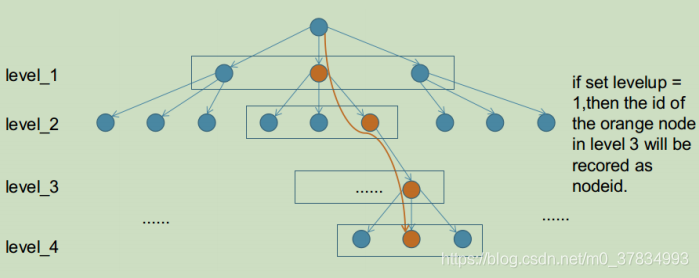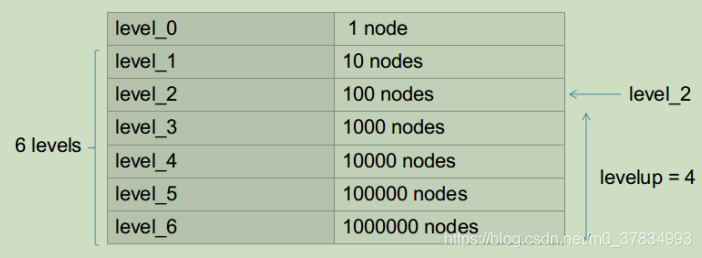其实处理非常简单, 在已经获得图像特征点集合的基础上, 再根据词典,对每个特征做一次分类. 再对第二幅图像提取特征, 然后也根据词典,也对这幅图像的所有特征进行分类.用分类后的特征类别代替原本的特征descriptor,即用一个数字代替一个向量进行比对, 显然速度可以大大提升。

### 正向索引，用于加速匹配

主要在以下几个函数

核心代码如下所示：

这种加速特征匹配的方法在ORB-SLAM2中被大量使用

• 正向索引的层数如果选择第0层（根节点），那么时间复杂度和暴力搜索一样
• 如果是叶节点层，则搜索范围有可能太小，错失正确的特征点匹配
• 作者一般选择第二层或者第三层作为父节点（L=6），正向索引的复杂度约为O(N2/Km)

### 逆向索引，用于回环和重定位

作者用反向索引记录每个叶节点对应的图像编号。当识别图像时，根据反向索引选出有着公共叶节点的备选图像并计算得分，而不需要计算与所有图像的得分。

使用词袋模型，在重定位过程中找出和当前帧具有公共单词的所有关键帧，在KeyFrameDatabase::DetectLoopCandidates()函数中代码如下：

# 改进

• 通过一些online learning的方法进行词典学习
• 把特征descriptor转为二进制表达, 加速运算
• 训练自己的特征

# 应用

• SLAM中的 回环检测

# Ref

]]>
<p>[TOC]</p> <h1 id="overview">Overview</h1> <h2 id="bag-of-words">Bag of Words</h2> <p><strong>BoW（Bag of Words，词袋模型）</strong>，是自然语言处理领域经常使
B-Spline for SLAM https://cgabc.xyz/posts/1022444e/ 2022-03-20T16:00:00.000Z 2022-08-26T12:03:32.808Z Overview

to convert a set of trajectory points into a continuous-timeuniform cubic cumulative b-spline

# Uniform Cubic B-Splines in$$\mathbb{SE(3)}$$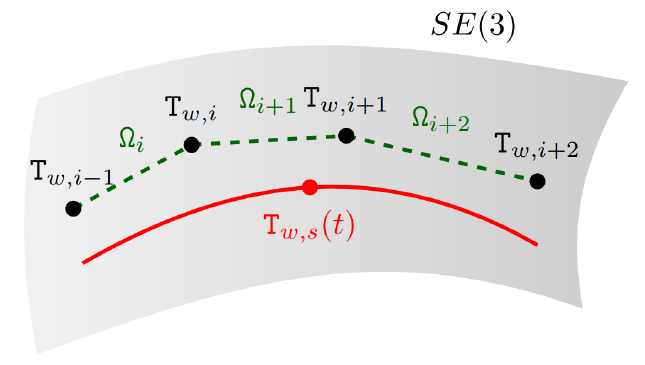# Continuous and Discrete Time

• 离散时间
• 通过IMU运动学模型，进行IMU预积分
• 跟Cam时间戳对齐
• 联合优化
• 连续时间
• Cam位姿BSpline拟合连续位姿
• 位姿微分

# Applications

## 连续时间轨迹估计

• https://jishuin.proginn.com/p/763bfbd6f25e

## IMU插值与数据仿真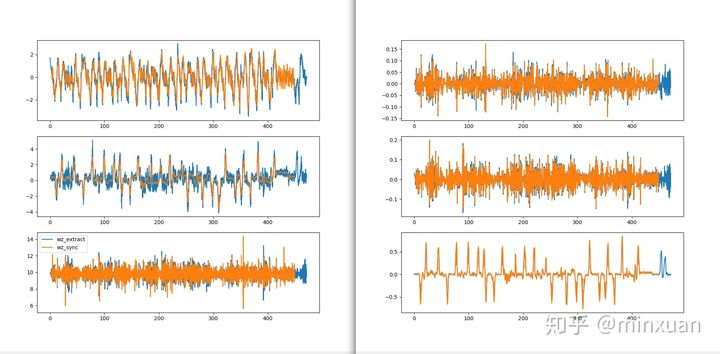### IMU数据仿真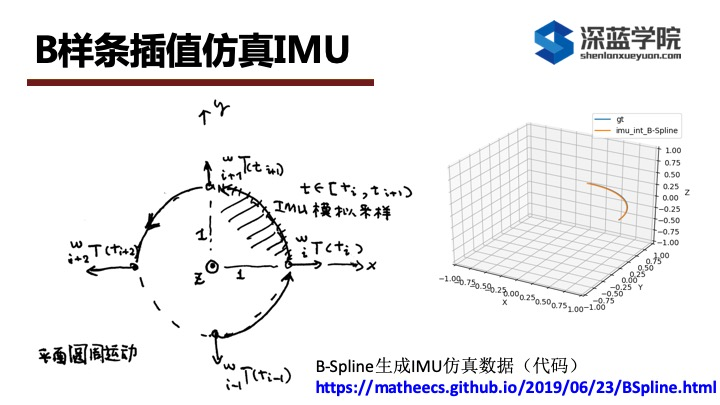## Unsynchronizedmulti-sensor intrinsic and extrinsic least-squares calibration

### IMU与Cam的相机外参标定

ref:

camera-imu外参标定大体上分为三步：

1. 粗略估计camera与imu之间时间延时。
• 利用camera的样条曲线获取任意时刻camera旋转角速度，而陀螺仪又测量imu的角速度
• 现在利用两个曲线的相关性，可以粗略估计imu和camera时间延时
2. 获取imu-camera之间初始旋转，还有一些必要的初始值：重力加速度、陀螺仪偏置。
3. 大优化，包括所有的角点重投影误差、imu加速度计与陀螺仪测量误差、偏置随机游走噪声。
]]>
<h1 id="overview">Overview</h1> <p>to convert a set of trajectory points into <strong>a continuous-time uniform cubic cumulative b-spline</s
TSDF Overview https://cgabc.xyz/posts/81f16034/ 2022-03-12T16:00:00.000Z 2022-08-26T12:03:32.800Z [TOC]

# Overview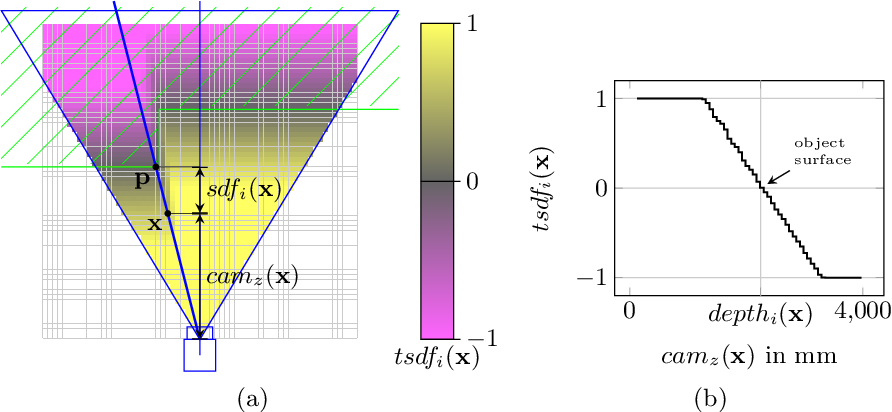SDF (Signed Distance Function)描述的是点到面的距离，在面上为0，在面的一边为正，另一边为负。

TSDF (Truncated SDF)只考虑面的邻域内的SDF值，邻域的最大值是maxtruncation的话，则实际距离会除以maxtruncation这个值，达到归一化的目的，所以TSDF的值在-1到+1之间。

# 算法逻辑TSDF模型将整个待重建的三维空间划分成网格，每个网格中存储了数值，网格模型中值的大小代表网格离重建好的表面的距离。

如下图表示的是重建的一个人的脸（网格模型中值为 0的部分，红线表示重建的表面，示意图给出的二维信息，实际是三维的），重建好的表面到相机一侧都是正值，另一侧都是负值，网格点离重建好的表面距离越远绝对值越大，在网格模型中从正到负的穿越点表示重建好的场景表面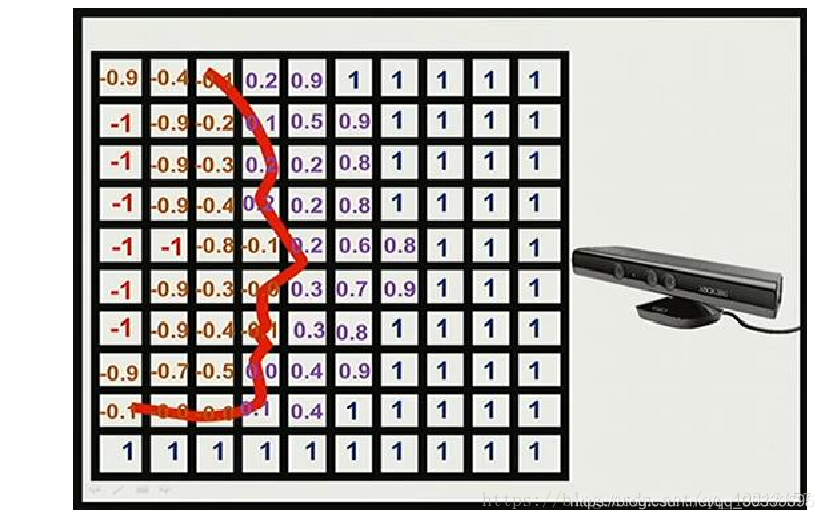我们将整个空间的体素全部存入GPU运算，每个线程处理一条(x,y)。即对于(x,y,z)的晶格坐标，每个GPU进程扫描处理一个(x,y)坐标下的晶格柱。

1. 对于每个x,y坐标下的体元g，并行的从前往后扫描

2. 将晶格坐标g转换到对应的世界坐标系点vg

3. 对于每次TSDF操作时的拍摄变换Ti反变换到对应的相机坐标系坐标v

4. 相机坐标系点v投影到图像坐标点p，从3D到2D

5. 如果v在此摄像机的投影范围内，用它修正现有tsdf表示

6. sdfi是该相机坐标系点vg到本次相机原点ti的距离与本次观测深度Di(p)的差值

7. 8-11为截断的过程，Truncated的意义所在，用maxtruncation表示选取的截断范围，此值将会关系到最后重建结果的精细程度

8. 如果差值为正，表示该晶格在本次测量的面的后面

9. tsdfi赋值【0,1】之间，越靠近观测面的地方值越接近0

10. 如果差值为负，表示该晶格在本次测量的面的前面

11. tsdfi赋值【-1，0】之间，越靠近观测面的地方值越接近0

12. 选取本次计算值的tsdf的权值wi，这个权值的选取直接关系到图片的适应性，以及抗噪声的能力，其实这里有点类似卡尔曼滤波。注意这里每次权值+1的操作基于这样的原因，由于只有在相机拍摄范围内的点才会进入求tsdf的操作，每次的权值在原先的基础上增加1能照顾到迅速变化的或很少扫描到的面的变化。

13. 加权平均求出tsdfavg

14. 将wi和tsdfavg存储在对应的晶格，进行下个晶格的扫描操作经过上面的扫描，最终立方体晶格中存储的tsdf值形成了重建物体外是负值，物体内部是正值，物体表面是0值得形式（可能没有准确的零值，但是可以根据正负值插值求出零值点，所以最后物体表面的分辨率将会超过晶格的分辨率）

# Example: MobileFusion

## 建立长方体包围盒，并划分网格

要建立一个长方体包围盒，让所有的三维点都在这个长方体里面。

假设z方向垂直相机，则x,y方向上的极值就是图像的边界。图像的边界点是就是四个角(0,0),(w,0),(0,h),(w,h)，z方向上深度范围是0~max_depth，组合而成的边界点就是（0，0，0），（0，0，max_depth）,(w,0,0)等的2^3=8种情况，然后把这些点用相机的内参和外参换算到世界坐标系中，长方体的极点。

在长方体内部划分网格，比如说我们现在求得的长方体的极点分别是（-1，-1，-1），（1，1，1），单位是米。我们要在这个长方体内部划分网格，就是分割出一个个等体积的小的立方体，也就是所谓的体素。我们让体素的边长是0.02，也就是2厘米。那么从-1到1，我们可以划分出100个体素，也就是说这个长方体上每个小立方体的8个顶点的坐标可以用（x,y,z）来表示，其中x,y,z都是0-100之间的，同时它们的世界坐标也可以通过（-1+0.02x,-1+0.02y,-1+0.02*z）来计算出来。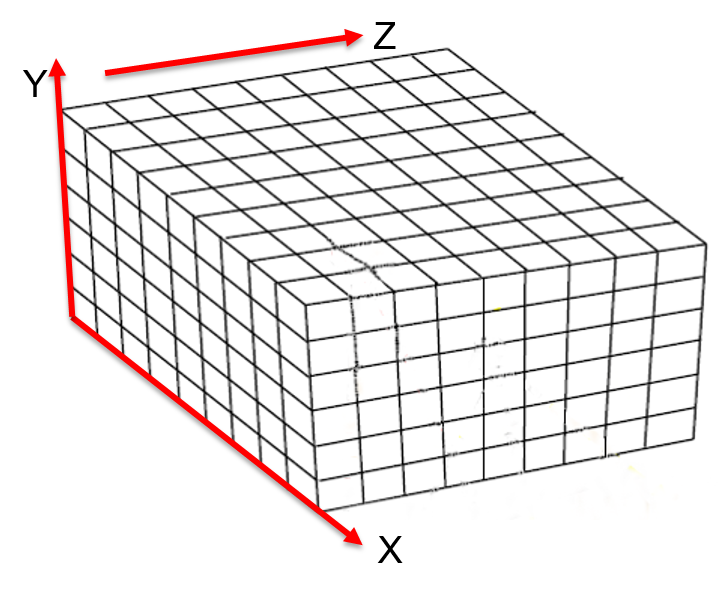## 迭代更新tsdf网格

遍历每一组数据（RGB图、深度图、pose.txt），每次把这个长方体内的所有格点的世界坐标通过逆变换到相机坐标，再投影到图片上。

将图片上对应位置的深度与格点的在相机坐标系下的深度比较

$\text{depth-diff} = \text{depth-val} - \text{cam-pts}[2,:]$

如果 $$\|\text{depth-diff}\| <\text{trunc-marin}$$ 则认为有效。

$$\text{dist} = \text{depth-diff} /\text{trunc-marin}$$ 去加权更新tsdf网格。

tsdf网格每个顶点存放的是dist的加权和。

## 找等值面

用marchingcubes算法在tsdf网格中寻找dist加权和为0的等值面，就是物体表面。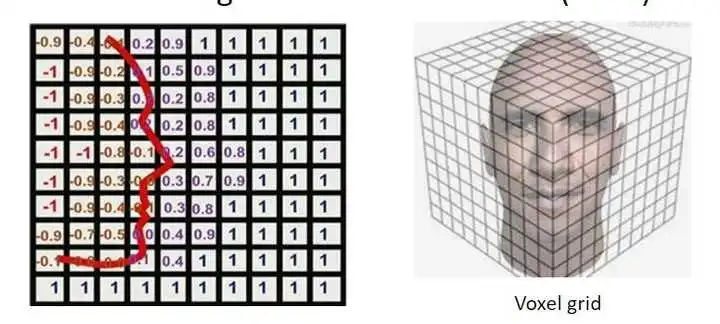# Related Projects

]]>
<p>[TOC]</p> <h1 id="overview">Overview</h1> <p align="center"> <img src="/img/post/map/sdf_tsdf.png" style="width:80%"> </p> <p><strong>SDF
EKF v.s. MSCKF v.s. MAP (VINS-Mono) https://cgabc.xyz/posts/dd7458d3/ 2022-03-07T16:00:00.000Z 2022-08-26T12:03:32.808Z [TOC]

# Overview

$z = f(x) + n, \quad n \sim \mathcal{N}(0, \Sigma), \quad z \sim\mathcal{N}(f(x), \Sigma)$

$P(z \mid x)= \mathcal{N}(z; f(x), \Sigma)= \eta \exp \left(-\frac{1}{2}(z-f(x))^{T} {\Sigma}^{-1}(z-f(x))\right)$

# EKF

true state

\begin{aligned}x_k &= f(x_{k-1}, w_{k-1}) \\z_k &= h(x_k, v_k)\end{aligned}

norminal state

\begin{aligned}\bar{x}_k &= f(\hat{x}_{k-1}, 0) \\\bar{z}_k &= h(\bar{x}, 0)\end{aligned}

true state linearization

\begin{aligned}x_k &\approx \bar{x}_k + A(x_{k-1} - \hat{x}_{k-1}) + W w_{k-1} \\z_k &\approx \bar{z}_k + H(x_k - \bar{x}_k) + V v_k\end{aligned}

prediction error & measurement residual

\begin{aligned}e_x &\equiv x_k - \bar{x}_k \approx A(x_{k-1} - \hat{x}_{k-1}) + Ww_{k-1} \\e_z &\equiv z_k - \bar{z}_k \approx H e_x + V v_k\end{aligned}

jacobian

$A = \frac{\partial f}{\partial x}, \quadW = \frac{\partial f}{\partial w}$

$H = \frac{\partial h}{\partial x}, \quadV = \frac{\partial h}{\partial v}$

covariance

$w \sim \mathcal{N}(0, Q), \quad v \sim \mathcal{N}(0, R)$

$e_x \sim \mathcal{N}(0, P) \rightarrow x \sim \mathcal{N}(\bar{x}, P),\quade_z \sim \mathcal{N}(0, S)$

$P = \texttt{cov}(e_x) = E(e_x e_x^T), \quad S = \texttt{cov}(e_z) =E(e_z e_z^T)$

## Prediction

state prediction (w/o noise)

$\hat{x}_k^- = f(\hat{x}_{k-1}, 0)$

(error state) covariance

$\text{cov}(x_k - \hat{x}_k^-) = P_k^- = A_k P_{k-1} A_k^T + W_k Q_{k-1}W_k^T$

## Update

Kalman gain

$K_k = P_k^- H_k^T S^{-1}, \quad S = H_k P_k^- H_k^T + V_k R_k V_k^T$

state update

$\hat{x}_k = \hat{x}_k^- + K_k (z_k - h(\hat{x}_k^-, 0))$

covariance update

$\text{cov}(x_k - \hat{x}_k) = P_k = (I - K_k H_k) P_k^-$

# MSCKF

## Prediction

### state prediction (stateprior)

$PVQ$

### propagate error cov P

continuous-time to discret-time，离散时间状态转移矩阵和噪声协方差矩阵 比较准确，例如

$F = \exp(A \Delta t) \approxI + A \Delta t + \frac{1}{2} (A \Delta t)^2 + \frac{1}{6} (A \Delta t)^3$

误差状态的概率分布

$\delta x \sim \mathcal{N}(\hat{\delta x}, P), \quad n \sim \mathcal{N}(0, Q)$

误差协方差传播（整个系统过程）

$\delta x_{i+1} = F \delta x_i + G n, \quad P = F P F^T + G Q G^T$

## Update (ESKF)

$\delta x \sim \mathcal{N}(\hat{\delta x}, P)$

$z = h(x) + v \approx h(x_0) + H \delta x + v, \quad v \sim\mathcal{N}(0, R)$

predicted residual (innovation)

$r = z - h(x_0) = H \delta x + v$

then, the covariance of innovation

$cov(r, r) = E(rr^T) = E(H \delta x \delta x^T H^T + vv^T) = HPH^T + R$

### update state and covariance

$x = K r$

$P = (I-KH)P$

# MAP (VINS-Mono)

## Prediction

### state prediction (stateprior)

$PVQ$

### pre-integration(propagate error cov P & state)

continuous-time to discret-time

$F = \exp(A \Delta t) \approx I + A \Delta t$

误差状态的概率分布

$\delta x \sim \mathcal{N}(0, P), \quad n \sim \mathcal{N}(0, Q)$

误差状态（状态预积分）和协方差传播（图像k时刻初始，图像k～图像k+1）

$\delta x_{i+1} = F \delta x_i + G n, \quad P = F P F^T + G Q G^T$

## Update (MAP)

### Jacobian & informationmatrix in MAP

$J = \frac{\partial r}{\partial \delta x}$

#### IMU

协方差矩阵（信息矩阵的逆）

$\text{cov}(\delta x_k) = P$

#### Cam

协方差矩阵（信息矩阵的逆）

$\text{cov}(r_k) = \Sigma_{\pi}$

### update state

$x = x + \delta x$

# QA

## Jacobi when Linear andNonlinear

欧式空间的非线性方程

$h(x) \approx h(x_0) + H \Delta x, \quad\left. H = \frac{\partial h(x)}{\partial x} \right|_{x = x_0}$

$$h(x)$$ 线性时

$h(x) = Hx$

## Jacobi w.r.t Error or TrueState

$f(x_0 \oplus \Delta x) = F(\Delta x)$

$f(x_0 \oplus \Delta x) \approx f(x_0) +\left. \frac{\partial f(x_0 \oplus \Delta x)}{\partial x}\right|_{x=x_0} \Delta x$

$F(\Delta x) \approx F(0) +\left. \frac{\partial F(\Delta x)}{\partial \Delta x} \right|_{\Delta x= 0} \Delta x =f(x_0) +\left. \frac{\partial f(x_0 \oplus \Delta x)}{\partial \Delta x}\right|_{\Delta x = 0} \Delta x$

当x在欧式空间时，上式等价。

• ref: https://zhuanlan.zhihu.com/p/75714471

### Jacobi in EKF & MAP

优化变量 是 什么状态，对应的 雅克比 即是 对什么状态 求导

#### EKF

w.r.t true statew.r.t error state
measurement function$$h(x)$$$$h(\Deltax)$$
Jacobi$$\frac{\partial h(x)}{\partial x}$$$$\frac{\partial h(x)}{\partial \Deltax}$$
init state$$x =x_0$$$${\Deltax}=0$$
update$$x \oplus\Delta x, \Delta x = Kr$$$$\Delta x =Kr$$

#### MAP

w.r.t true-statew.r.t error-state
cost function$$f(x)$$$$f(\Deltax)$$
Jacobi$$\frac{\partial f(x)}{\partial x}$$$$\frac{\partial f(x)}{\partial \Deltax}$$
init state$$x =x_0$$$${\Deltax}=0$$
iteration update$$x \oplus\delta x$$$$\Delta x\oplus \delta \Delta x$$
]]>

• 3 DoF

ref:

## XYZ

• Global XYZ
• Anchored XYZ

## Inverse Depth

• Global inverse depth (spherical coordinates)
• has a singularity when the z-distance goes to zero
• 球坐标逆深度仅在xyz都趋近于0时才存在数值奇异，所以能用在全局坐标系
• Anchored inverse depth (MSCKF)
• 逆深度 + normalized UV (Bearing Vector)
• Anchored inverse depth (MSCKF single depth)
• 逆深度
• the the single depth from VINS-Mono

# 3D Line

$L: \left[ P_0 \; P_1 \right]$

• 4 DoF

ref:

• 过参数化 问题

# 3D Plane

$Ax + By + Cz + D = 0$

• 3 DoF

ref:

## Hesse

用一个平面的 单位法向量 和 平面距离原点的距离 来表示

$\pi: \left(\mathbf{n}^{\top}, d\right)^{\top} \in \mathbb{R}^4$

过参数化问题：平面的Hesse形式的过参数化就是因为单位法向量部分有3个参数，但是实际只有两个自由度导致的。

## 球坐标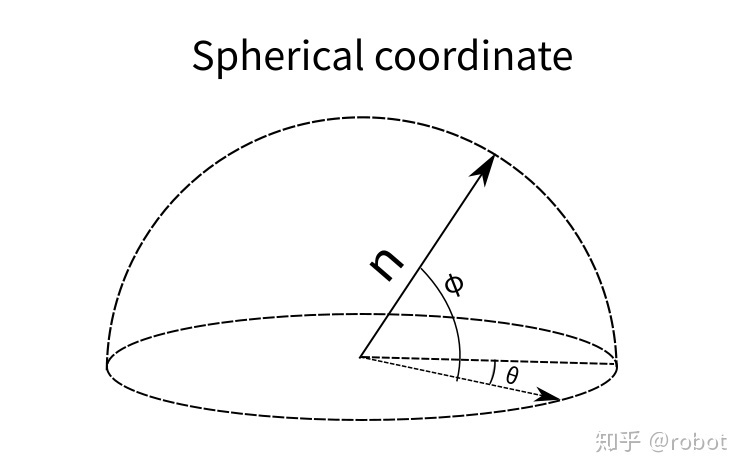单位法向量可以被看成是一个单位圆球上的一点，那么就可以用两个角度$$\theta$$$$\phi$$来参数化这个点，从而表示出这个单位法向量。

$\pi: \left[ \theta \; \phi \; d \right] \in \mathbb{R}^3$

$\tau=(\theta, \phi, d)^{\top}=q(\pi)=\left(\theta=\arctan \frac{n_{y}}{n_{x}}, \; \phi=\arcsin n_{z},\; d\right)^{\top}$

## 切平面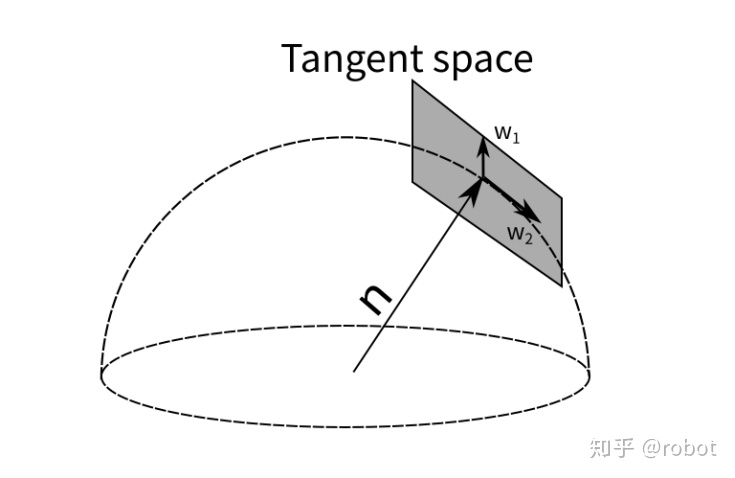GTSAM里面表示面的方式用切平面的方式来更新单位法向量，同样也可以被用来优化单位法向量。

## 最近点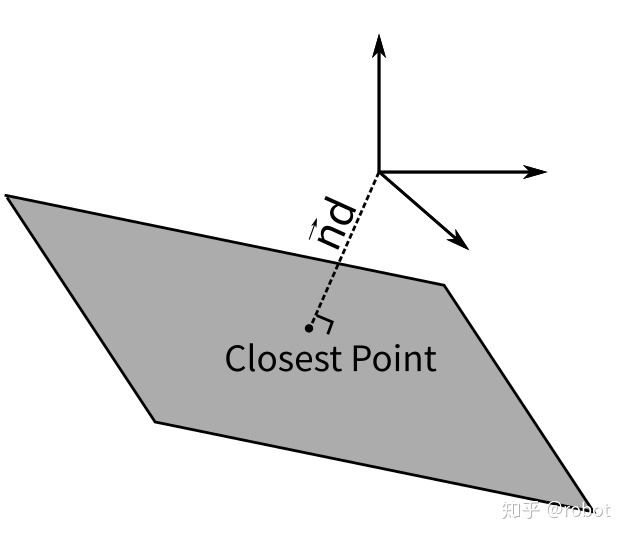# Unified Representation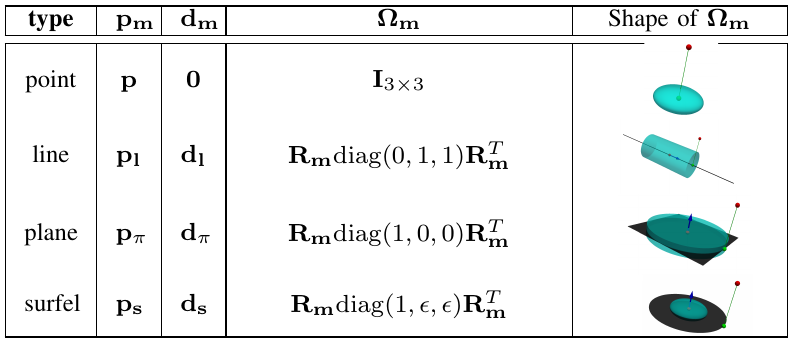# Reference

1. Unified Representation ofHeterogeneous Sets of Geometric Primitives↩︎
]]>
<p>[TOC]</p> <h1 id="overview">Overview</h1> <ul> <li><p>一般表示形式</p></li> <li><p>优化形式（避免过参数化）</p></li> </ul> <p>特征的参数化表示，即以何种方式表示特征，在优化中决定了特征
基于RGBD相机的多模态结构特征融合定位 (Draft) https://cgabc.xyz/posts/ec177663/ 2022-03-05T16:00:00.000Z 2022-08-26T12:03:32.804Z [TOC]

# 概述

目前基于RGBD相机的视觉SLAM系统，都是基于特征点或者稠密点云进行定位，计算量大，而且在无纹理或弱纹理的环境，容易跟丢，鲁棒性较差。本文提出了一种基于RGBD相机的多模态结构化数据融合定位方法，算法主要框架如下图所示，通过充分利用视觉特征，融合二维特征点、二维曲线（边缘点）、二维线段和三维平面等，结合状态估计算法进行实时、鲁邦、准确的位姿估计。在二维特征点的基础上，同时提取边缘特征，使其在弱纹理的环境也能快速、鲁棒定位；另外，在每个关键帧提取2D线段和3D平面等特征，在不增加较多计算量的情况下，引入更多稳定的视觉约束，使位姿估计更准确。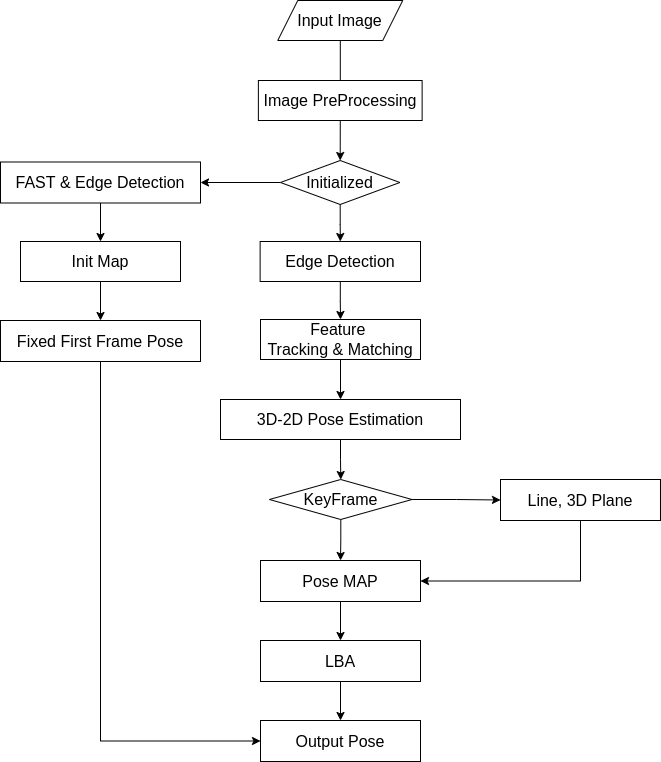# 初始化

（1）在第一帧图像提取FAST特征点和进行边缘检测

（2）根据深度图，通过相机投影模型，得到对应的3D路标点，创建初始地图

（3）固定第一帧位姿为单位阵

# 数据降维

我们将输入的图像（灰度图和深度图），进行特征提取，获取一些不变的特征，为后面的状态估计提供数据支持。本算法用到的视觉特征主要包括以下四种：

• 2D特征点
• 线
• 2D线段
• 2D曲线或边缘点
• 3D平面

## 特征提取

### 2D图像特征检测

• 2D特征点：FAST / ORB
• 2D线段：LSD
• 2D曲线或边缘点：Canny边缘检测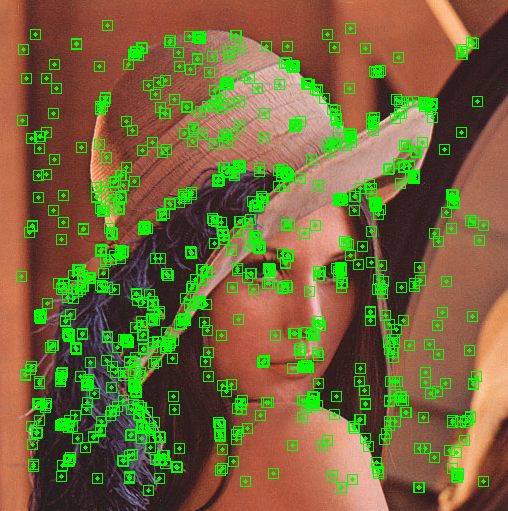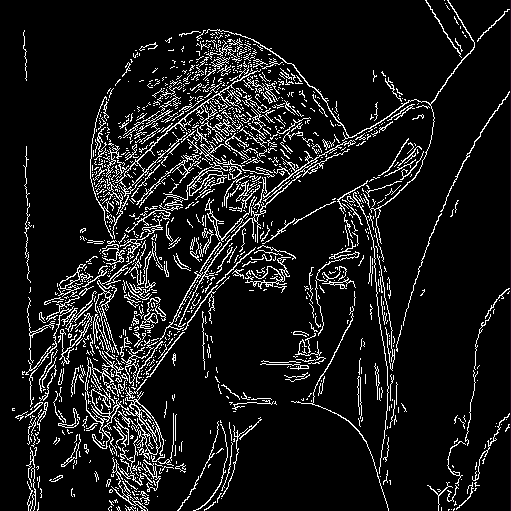### 3D点云平面提取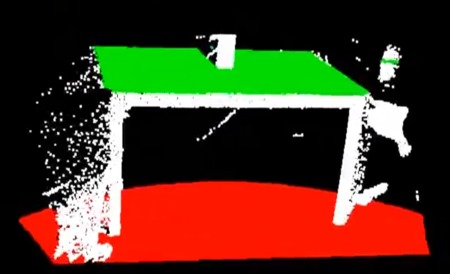3D平面特征是SLAM系统中减小漂移误差的一种稳定标志。从密集点云中提取平面是一种简单的方法，常用于RGB-D相机或激光雷达。但基于稠密点云提取平面，算力较高，不利于SLAM定位的实时性；我们利用RANSAC方法从上面得到的稀疏路标点中提取3D平面。

（1）根据2D特征点和2D边缘点对应的路标点（见下面三角化章节）作为输入的3D稀疏点云

（2）随机选取三个不共线的点，计算平面参数（质心和法向量，其表示可见下面3D路标表示章节）

（3）计算稀疏点云中每个点到平面的距离，并累加，记录距离累加和、平面参数

（4）重复步骤（2）~（3），获取距离累加和最小时的平面参数

（5）收集内点：计算所有点到该平面的距离，并设定阈值，小于阈值的所有点即为平面点内点

（6）计算所有内点的均值为最终平面的 质心

（7）根据均值计算其协方差矩阵，对该矩阵进行奇异值分解，对应奇异值最小的奇异向量即为最终平面的法向量

example code:

# 数据关联

我们在提取了2D特征后，需要通过2D特征间的匹配，三角化得到3D路标，并与对应的2D特征进行关联；同时，为了兼顾实时性和高性能，还要提取关键帧。

## 2D特征匹配

• 2D特征点：光流跟踪 / ORB描述子匹配
• 2D线段：LBD描述子匹配
• 2D曲线或边缘点（点云）：通过 距离变换 进行倒角匹配
• 3D平面：根据两平面参数（法向量和质心），计算法向量之间的夹角以及质心间的欧式距离，作为平面匹配的相似度，据此进行匹配

## 3D路标及其参数化表示

### 3D路标点

$P = [X, Y, Z]^T$

（1）2D特征点和2D边缘点对应的3D路标点，初始在深度图中提取

（2）因深度图距离限制、空洞（深度缺失）以及3D路标点质量较差被剔除等因素，需要后续补充3D路标点，通过对极几何的极线搜索算法，三角化出3D路标点，如下图所示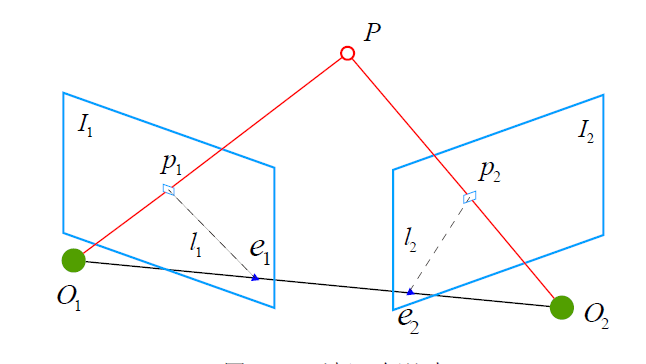### 3D直线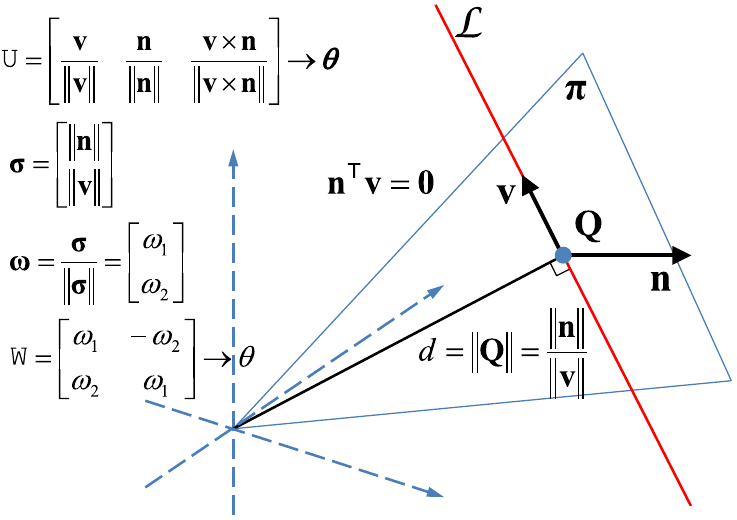3D空间直线有4个自由度，其表示方式主要有两种：普吕克坐标和正交表示。

普吕克坐标

$\mathcal{L}=\left(\mathbf{n}^{\top}, \mathbf{v}^{\top}\right)^{\top}\in \mathbb{R}^6$

正交表示

$(\mathrm{U}, \mathrm{W}) \in S O(3) \times S O(2)\longrightarrow\left[ \boldsymbol{\theta} \; \theta \right] \in \mathbb{R}^4$

通常，为方便表示空间直线，我们使用普吕克坐标；但由于其具有6个变量，多于空间直线的4个自由度，在优化时会出现过参数化问题，因此优化中使用其正交表示。

通过在两个视图图像中提取到的2D线段进行匹配，线段匹配对三角化出空间直线（普吕克坐标表示），并与2D线段进行关联。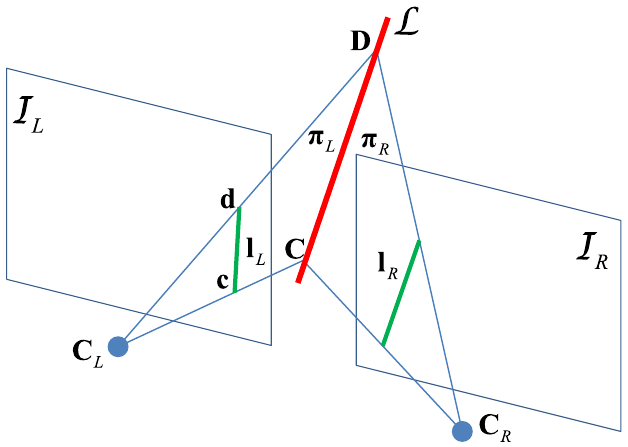### 3D平面

欧式空间3D平面有3个自由度，在本算法中对其表示方式主要有两种：Hesse形式、球坐标。

Hesse形式$\pi=\left(\mathbf{n}^{\top}, d\right)^{\top} \in \mathbb{R}^4$

球坐标$\tau=(\theta, \phi, d)^{\top}=q(\pi)=\left(\theta=\arctan \frac{n_{y}}{n_{x}}, \; \phi=\arcsin n_{z},\; d\right)^{\top}$

一般优化中采用球坐标的表示方式

## 关键帧选取

主要根据时空两大方面，对关键帧进行选取。

（1）时间：经过一定数量的帧数

（2）空间：当当前帧距离上一关键帧的位姿变换超过一定阈值

# 状态估计

## 初始位姿估计

利用2D点特征和3D路标点，通过PNP算法，进行初始位姿估计。

## 后端优化

优化变量为当前帧相机位姿

$X =\left[ t_x, t_y, t_z, \theta_x, \theta_y, \theta_z \right]^T$

定义总体目标函数如下

${X}^{*} =\arg \min_{X}\left\{\sum \rho\left(\left\|\mathbf{r}_{point}\right\|^{2}\right)+\sum \rho\left(\left\|\mathbf{r}_{edge} \right\|^2 \right)+\sum \rho\left(\left\|\mathbf{r}_{line} \right\|^2 \right)+\sum \rho\left(\left\|\mathbf{r}_{plane} \right\|^2 \right)\right\}$

### 特征点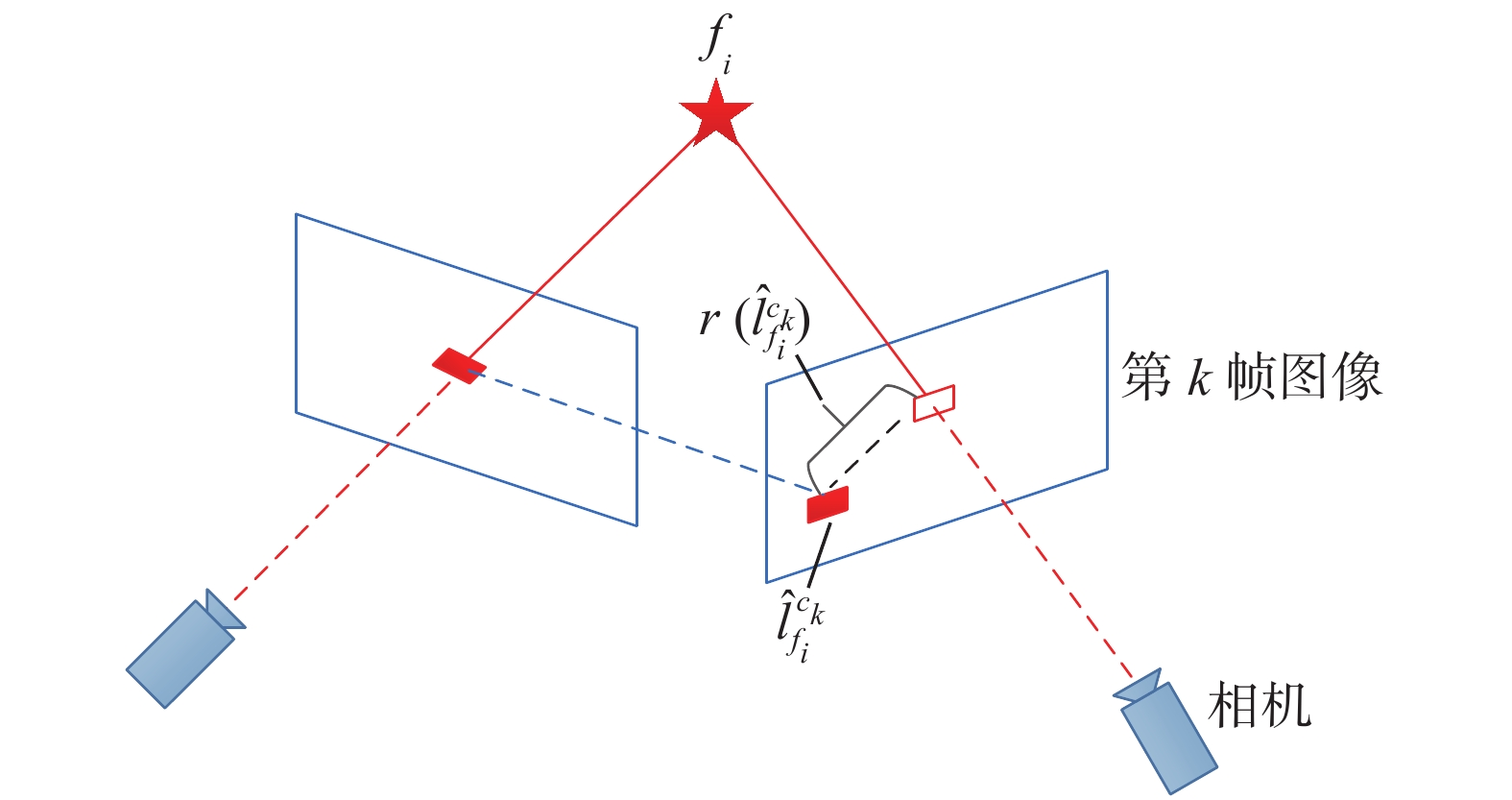（1）通过FAST或者光流法跟踪在参考关键帧图像上得到2D特征点，并得到与其关联的3D路标点。

（2）根据当前帧与参考帧间的位姿，将上述3D路标点投影到当前帧图像上，作为投影预测值。

（3）在当前帧图像上检测到的2D特征点中，找到与参考帧匹配的2D特征点，作为观测值。

（4）计算观测特征点到投影点的欧式距离，即重投影误差，作为观测残差。

残差定义为

$r_{point} = u - K \cdot T_{cr} P_r$

### 线段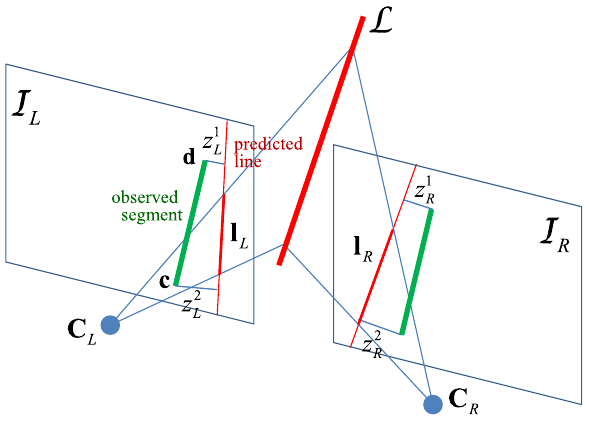（1）通过LSD算法在参考关键帧图像上提取到2D线段特征，并得到与其关联的3D空间直线。

（2）根据当前帧与参考帧间的位姿，将上述3D空间直线投影到当前帧图像上，作为投影预测值。

（3）在当前帧图像上检测到的2D线段中，找到与参考帧匹配的2D线段，作为观测值。

（4）计算观测线段两个端点到投影直线的欧式距离，即线重投影误差，作为观测残差。

残差定义为

$r_{line} = D(z, L, T_{cw})$

### 曲线或边缘点（ICP）

通过Canny边缘检测算法，在参考帧 $$F_{ref}$$ 中检测到2D边缘点 $$\mathbf{p}_{i}^{\mathcal{F}_{\text{ref}}}$$ ，根据相机投影模型和深度图像，得到 3D边缘点，如下

$\mathcal{S}^{\mathcal{F}_{\text {ref}}}=\left\{\mathbf{s}_{i}^{\mathcal{F}_{\text {ref}}}\right\}=\left\{d_{i}^{\mathcal{F}_{\text {ref }}}\pi^{-1}\left(\mathbf{p}_{i}^{\mathcal{F}_{\text {ref }}}\right)\right\}$

根据当前帧与参考帧间的位姿，将上述3D点投影到当前帧 $$F_k$$，得到2D点 $$\mathbf{o}_{i}^{\mathcal{F}_{k}}$$，如下

$\mathcal{O}^{\mathcal{F}_{k}}=\left\{\mathbf{o}_{i}^{\mathcal{F}_{k}}\right\}=\left\{\pi\left(\mathbf{R}^{\mathrm{T}}\left(\mathbf{s}_{i}^{\mathcal{F}_{\text{ref }}}-\mathbf{t}\right)\right)\right\}$

在当前帧检测到的2D点 $$P^{F_K}$$中找到 $$\mathbf{o}_{i}^{\mathcal{F}_{k}}$$的最近邻点，定义公式如下

$n\left(\mathbf{o}_{i}^{\mathcal{F}_{k}}\right)=\underset{\mathbf{p}_{j}^{\mathcal{F}_{k}}\in\mathcal{P}^{\mathcal{F}_{k}}}{\operatorname{argmin}}\left\|\mathbf{p}_{j}^{\mathcal{F}_{k}}-\mathbf{o}_{i}^{\mathcal{F}_{k}}\right\|$

残差定义为

$r_{edge} =\mathbf{o}_{i}^{\mathcal{F}_{k}}-n\left(\mathbf{o}_{i}^{\mathcal{F}_{k}}\right)$

定义目标函数

$X^*=\underset{X}{\operatorname{argmin}}\sum_{i=1}^{N}\left\|\mathbf{o}_{i}^{\mathcal{F}_{k}}-n\left(\mathbf{o}_{i}^{\mathcal{F}_{k}}\right)\right\|^{2}$

### 平面

（1）通过RANSAC算法在参考关键帧提取世界坐标系的3D平面。

（2）根据参考帧的位姿 $$T_{rw}$$以及当前帧与参考帧间的位姿 $$T_{cr}$$，将上述3D平面投影到当前帧，作为预测值。

（3）在当前帧提取3D平面，找到与参考帧匹配的3D平面，作为观测值。

（4）计算观测值与预测值之间的误差，作为观测残差。

残差定义为

$r_{plane} =q(\pi_c^w) -q(T_{cr}^{-1} T_{rw}^{-1} \pi_r^w)$

]]>
<p>[TOC]</p> <h1 id="概述">概述</h1> <p>目前基于RGBD相机的视觉SLAM系统，都是基于特征点或者稠密点云进行定位，计算量大，而且在无纹理或弱纹理的环境，容易跟丢，鲁棒性较差。本文提出了一种基于RGBD相机的多模态结构化数据融合定位方法，算法主要框